Abstract

We present a general formulation for incompressible fluid flow analysis using the finite element method (FEM). The standard Eulerian formulation is described first. The necessary stabilization for dealing with convective effects and the incompressibility condition are introduced via the Finite Calculus (FIC) method. A simple extension of the fluid flow equations to an arbitrary Lagrangian-Eulerian (ALE) frame adequate for treating fluid-structure interaction problems is briefly presented. A fully Lagrangian formulation called the Particle Finite Element Method (PFEM) is also described. The PFEM is particularly attractive for fluid-structure interaction problems involving large motions of the free surface and breaking waves. Examples of application of the Eulerian, the ALE and the fully lagrangian PFEM formulations are presented.

Keywords: Stabilized formulation, incompressible fluid, finite calculus, finite element method, particle finite element method, fluid-structure interaction, lagrangian flow.

1 INTRODUCTION

The development of efficient and robust numerical methods for analysis of incompressible flows has been a subject of intensive research in the last decades. Much effort has been spent in developing the so called stabilized numerical methods overcoming the two main sources of instability in incompressible flow analysis, namely those originated by the high values of the convective terms and those induced by the difficulty in satisfying the incompressibility condition.

The solution of above problems in the context of the finite element method (FEM) has been attempted in a number of ways . The underdiffusive character of the Galerkin FEM for high convection flows (which incidentaly also occurs for the central finite difference (FD) and finite volume (FV) methods ) has been corrected by adding some kind of artificial viscosity terms to the standard Galerkin equations.

A popular way to overcome the problems with the incompressibility constraint is by introducing a pseudo-compressibility in the flow and using implicit and explicit algorithms developed for this kind of problems such as artificial compressibility schemes  and preconditioning techniques . Many FEM schemes for fluid flow analysis with good stabilization properties for the convective and incompressibility terms are based in Petrov-Galerkin (PG) techniques. The background of PG methods are the non-centred (upwind) schemes for computing the first derivatives of the convective operator in FD and FV methods . A general class of Galerkin FEM has been recently developed where the standard Galerkin variational form of the momentum and mass balance equations is extended with adequate residual-based terms in order to achieve a stabilized numerical scheme. Among the many finite element methods of this kind we can name the Streamline Upwind Petrov Galerkin (SUPG) method , the Galerkin Least Square (GLS) method , the Taylor-Galerkin method , the Characteristic Galerkin method  and its variant the Characteristic Based Split (CBS) method , the pressure gradient operator method  and the Subgrid Scale (SS) method . A good review of these methods can be found in .

In this paper a stabilized finite element formulation for incompressible flows is derived in a different manner. The starting point is the modified governing differential equations of the fluid flow problem formulated via a finite calculus (FIC) approach . The FIC method is based in invoking the balance of fluxes in a fluid domain of finite size. This introduces naturally additional terms in the classical differential equations of momentum and mass balance of infinitesimal fluid mechanics which are a function of the balance domain dimensions. The new terms in the modified governing equations provide the necessary stabilization to the discrete equations obtained via the standard Galerkin FEM. The resulting stabilized formulation overcomes the problems due to the convective and incompressibility terms and it allows the use of low order finite elements (such as linear triangles and tetrahedra) with equal order approximations for the velocity and pressure variables 33. Application of the FIC approach to the solution of fluid flow problems using a meshless methods are presented in .

The layout of the paper is the following. In the next section the main concepts of the FIC approach are introduced via a simple 1D convection-diffusion model problem. Then the basic FIC equations for incompressible flow problems formulated in an Eulerian frame are presented. The finite element discretization is introduced and the resulting matrix equations are detailed. A fractional step scheme and a predictor-corrector scheme for the transient solution are presented.

The Eulerian formulation is extended to account for free surface wave effects by using an arbitrary Eulerian-Lagrangian (ALE) description and introducing the free surface boundary conditions. The numerical treatment of the free surface equation using the FIC method is presented. The analysis of fluid-structure interaction problems involving the movement of floating or submerged solids in a fluid is also discussed. These problems require to follow the displacement of the mesh nodes accordingly to the motion of the structure or the free surface and here a simple and effective algorithm for updating the mesh nodes is described.

In the last part of the paper the fully Lagrangian formulation for fluid flow analysis is presented as a particular case of the ALE form. More specifically, in this paper we describe an innovative Lagrangian formulation to solve problems involving the interaction between fluids and solids in a unified manner. The procedure, called the Particle Finite Element Method (PFEM) , treats the mesh nodes in the fluid and solid domains as dimensionless particles which can freely move an even separate from the main fluid domain representing, for instance, the effect of water drops. A finite element mesh connects the nodes defining the discretized domain where the governing equations are solved in the standard FEM fashion. The PFEM has many advantages for tracking the displacement of fluid particles in flows where large motions of the fluid surface occur such in the case of breaking waves, splashing of water, filling of moulds, etc.

The main advantage of the Lagrangian flow formulation is that the convective terms do not enter into the fluid equations. The difficulty is however transferred to the problem of adequately (and efficiently) moving the mesh nodes. We use a mesh regeneration procedure blending elements of different shapes based on an extended Delaunay tesselation .

The examples show the efficiency of the Eulerian, ALE and PFEM formulations to solve classical fluid flow problems, as well as more complex fluid-structure interaction problems involving contact with moving solids, waves around ships and large motions of the free surface, among others.

2 THE FINITE CALCULUS METHOD

We will consider a convection-diffusion problem in a 1D domain ${\textstyle \Omega }$ of length ${\textstyle L}$. The equation of balance of fluxes in a subdomain of size ${\textstyle d}$ belonging to ${\textstyle \Omega }$ (Figure 1) is written as

 $q_{A}-q_{B}=0$
(1)

where ${\textstyle q_{A}}$ and ${\textstyle q_{B}}$ are the incoming and outgoing fluxes at points ${\textstyle A}$ and ${\textstyle B}$, respectively. The flux ${\textstyle q}$ includes both convective and diffusive terms; i.e. ${\textstyle q=u\phi -k{d\phi \over dx}}$, where ${\textstyle \phi }$ is the transported variable, ${\textstyle u}$ is the velocity and ${\textstyle k}$ is the diffusitivity of the material.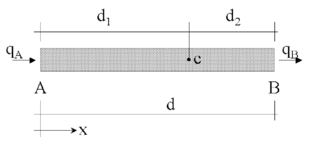Figure 1: Equilibrium of fluxes in a balance domain of finite size

Let us express now the fluxes ${\textstyle q_{A}}$ and ${\textstyle q_{B}}$ in terms of the flux at an arbitrary point ${\textstyle C}$ within the balance domain (Figure 1). Expanding ${\textstyle q_{A}}$ and ${\textstyle q_{B}}$ in Taylor series around point ${\textstyle C}$ up to second order terms gives after simplification

 ${\frac {dq}{dx}}-{\underline {{\frac {h}{2}}{\frac {d^{2}q}{dx^{2}}}}}{\frac {h}{2}}{\frac {d^{2}q}{dx^{2}}}=0$
(2)

where ${\textstyle h=d_{1}-d_{2}}$ and all the derivatives are computed at point ${\textstyle C}$.

Standard calculus theory assumes that the domain ${\textstyle d}$ is of infinitesimal size and the resulting balance equation is simply ${\textstyle {dq \over dx}=0}$. The new balance equation (2) derived assuming that the balance domain has a finite size, incorporates now the underlined term which introduces the characteristic length ${\textstyle h}$. Distance ${\textstyle h}$ can be interpreted as a free parameter depending on the location of point ${\textstyle C}$ (note that ${\textstyle -d\leq h\leq d}$). Eq.(2) is the starting point to derive numerical schemes with enhanced stability properties simply by computing the characteristic length parameter from an adequate “optimality” rule.

Consider, for instance, the modified equation (2) applied to the convection-diffusion problem. Neglecting third order derivatives of ${\textstyle \phi }$, eq.(3) can be written in an explicit form as

 $-u{\frac {d\phi }{dx}}+\left(k+{\frac {uh}{2}}\right){\frac {d^{2}\phi }{dx^{2}}}=0$
(3)

We see that the modified equation via the FIC method introduces naturally an additional diffusion term into the standard convection-diffusion equation. This is the basis of the popular “artificial diffusion” procedure [1,2,8,32, 36]. In the discretized problem, the characteristic length ${\textstyle h}$ is typically expressed as a function of the cell or element dimensions. The critical and optimal values of ${\textstyle h}$ for each cell or element can be computed from numerical stability conditions such as obtaining a physically meaningful solution, or even obtaining “exact” nodal values .

Equation (3) can be extended to account for source effects. The FIC equation can be then written in compact form as

 $r-{\underline {{\frac {h}{2}}{\frac {dr}{dx}}}}{\frac {h}{2}}{\frac {dr}{dx}}=0$
(4)

with

 $r=-u{\frac {d\phi }{dx}}+{\frac {d}{dx}}\left(k{\frac {d\phi }{dx}}\right)+Q$
(5)

where ${\textstyle Q}$ is the external source. A consistent “finite” form of the Neumann boundary condition can be obtained by invoking balance of fluxes in a domain of finite size next to the boundary ${\textstyle \Gamma _{q}}$ where the external (diffusive) flux is prescribed to a value ${\textstyle q_{p}}$. The modified Neumann boundary condition in the FIC method is 

 $k{\frac {d\phi }{dx}}+q_{p}-{\underline {{\frac {h}{2}}r}}{\frac {h}{2}}r=0\quad {\hbox{at }}\,\Gamma _{q}$
(6)

The definition of the problem is completed with the standard Dirichlet condition prescribing the value of ${\textstyle \phi }$ at the boundary ${\textstyle \Gamma _{\phi }}$.

The underlined terms in Eqs.(4) and (6) introduce the necessary stabilization in the discrete solution of the problem using whatever numerical scheme. For details see .

The time dimension can be introduced in the FIC method by considering the balance equation in a space-time slab domain .

The starting point in the next section are the FIC equations for a viscous incompressible fluid accounting for space stabilization terms only.

3 GENERAL FIC EQUATIONS FOR VISCOUS INCOMPRESSIBLE FLOW

The FIC governing equations for a viscous incompressible fluid can be written in an Eulerian frame of reference as

Momentum

 $r_{m_{i}}-{\underline {{1 \over 2}h_{j}{\partial r_{m_{i}} \over \partial x_{j}}}}{1 \over 2}h_{j}{\partial r_{m_{i}} \over \partial x_{j}}=0\qquad {\hbox{in }}\Omega$
(7)

Mass balance

 $r_{d}-{\underline {{1 \over 2}h_{j}{\partial r_{d} \over \partial x_{j}}}}{1 \over 2}h_{j}{\partial r_{d} \over \partial x_{j}}=0\qquad {\hbox{in }}\Omega$
(8)

where

 $r_{m_{i}}=\rho \left({\partial u_{i} \over \partial t}+u_{j}{\partial u_{i} \over \partial x_{j}}\right)+{\partial p \over \partial x_{i}}-{\partial s_{ij} \over \partial x_{j}}-b_{i}$ (9) $r_{d}={\partial u_{i} \over \partial x_{i}}\qquad i,j=1,n_{d}$ (10)

Above ${\textstyle \Omega }$ is the analysis domain, ${\textstyle n_{d}}$ is the number of space dimensions (${\textstyle n_{d}=2}$ for 2D problems), ${\textstyle u_{i}}$ is the velocity along the ith global axis, ${\textstyle \rho }$ is the (constant) density of the fluid, ${\textstyle p}$ is the absolute pressure (defined positive in compression), ${\textstyle b_{i}}$ are the body forces and ${\textstyle s_{ij}}$ are the viscous deviatoric stresses related to the viscosity ${\textstyle \mu }$ by the standard expression

 $s_{ij}=2\mu \left({\dot {\varepsilon }}_{ij}-\delta _{ij}{1 \over 3}{\partial u_{k} \over \partial x_{k}}\right)$
(11)

where ${\textstyle \delta _{ij}}$ is the Kronecker delta and the strain rates ${\textstyle {\dot {\varepsilon }}_{ij}}$ are

 ${\dot {\varepsilon }}_{ij}={1 \over 2}\left({\partial u_{i} \over \partial x_{j}}+{\partial u_{j} \over \partial x_{i}}\right)$
(12)

The FIC boundary conditions are

 $n_{j}\sigma _{ij}-t_{i}+{\underline {{1 \over 2}h_{j}n_{j}r_{m_{i}}}}{1 \over 2}h_{j}n_{j}r_{m_{i}}=0\quad {\hbox{on }}\Gamma _{t}$
(13)
 $u_{j}-u_{j}^{p}=0\quad {\hbox{on }}\Gamma _{u}$
(14)

and the initial condition is ${\textstyle u_{j}=u_{j}^{0}}$ for ${\textstyle t=t_{0}}$.

Summation convention for repeated indices in products and derivatives is used unless otherwise specified.

In Eqs.(13) and (14) ${\textstyle t_{i}}$ and ${\textstyle u_{j}^{p}}$ are surface tractions and prescribed displacements on the boundaries ${\textstyle \Gamma _{t}}$ and ${\textstyle \Gamma _{u}}$, respectively, ${\textstyle n_{j}}$ are the components of the unit normal vector to the boundary and ${\textstyle \sigma _{ij}}$ are the total stresses given by ${\textstyle \sigma _{ij}=s_{ij}-\delta _{ij}p}$. The sign in front the stabilization term in Eq.(13) is positive due to the definition of ${\textstyle r_{m_{i}}}$ in Eq.(9).

The ${\textstyle h_{i}'s}$ in above equations are characteristic lengths of the domain where balance of momentum and mass is enforced. In Eq.(13) these lengths define the domain where equilibrium of boundary tractions is established . In the discretized problem the ${\textstyle h_{i,s}}$ become of the order of a typical element dimension as described in Section 9. Note that by making ${\textstyle h_{i}=0}$ the standard infinitessimal form of the fluid mechanics equations is recovered .

Eqs.(7)–(14) are the starting point for deriving stabilized FEM for solving the incompressible Navier-Stokes equations. The underlined FIC terms in Eq.(7) are essential to overcome the numerical instabilities due to the convective terms in the momentum equations, whereas the underlined terms in Eq.(8) take care of the instabilities due to the incompressibility constraint. An interesting feature of the FIC formulation is that it allows to use equal order interpolation for the velocity and pressure variables .

3.1 Stabilized integral forms

From the momentum equations it can be obtained 

 ${\partial r_{d} \over \partial x_{i}}\simeq {h_{j} \over 2a_{i}}{\partial r_{m_{i}} \over \partial x_{j}}\quad ,\quad {\hbox{no sum in }}i$
(15)

where

 $a_{i}={2\mu \over 3}+{\rho u_{i}h_{i} \over 2}\quad ,\quad {\hbox{no sum in }}i$
(16)

Substituting Eq.(15) into Eq.(8) and retaining the terms involving the derivatives of ${\textstyle r_{m_{i}}}$ with respect to ${\textstyle x_{i}}$ only, leads to the following alternative expression for the stabilized mass balance equation

 $r_{d}-\sum \limits _{i=1}^{n_{d}}\tau _{i}{\partial r_{m_{i}} \over \partial x_{i}}=0$
(17)

with

 $\tau _{i}=\left({8\mu \over 3h_{i}^{2}}+{2\rho u_{i} \over h_{i}}\right)^{-1}$
(18)

The ${\textstyle \tau _{i}}$'s in Eq.(18) when scaled by the density are termed in the stabilization literature intrinsic time parameters. The interest of Eq.(17) is that it introduces the first space derivatives of the momentum equations (and the corresponding laplacian of pressure terms) into the mass balance equation. These terms have intrinsic good stability properties as explained next.

The weighted residual form of the momentum and mass balance equations (Eqs.(7) and (17)) is written as

 $\int _{\Omega }\delta u_{i}\left[r_{m_{i}}-{h_{j} \over 2}{\partial r_{m_{i}} \over \partial x_{j}}\right]+\int _{\Gamma _{t}}\delta u_{i}(\sigma _{ij}n_{j}-t_{i}+{h_{j} \over 2}n_{j}r_{m_{i}})d\Gamma =0$
(19)
 $\int _{\Omega }q\left[r_{d}-\sum \limits _{i=1}^{n_{d}}\tau _{i}{\partial r_{m_{i}} \over \partial x_{i}}\right]d\Omega =0$
(20)

where ${\textstyle \delta u_{i}}$ and ${\textstyle q}$ are arbitrary weighting functions representing virtual velocities and virtual pressure fields. Integrating by parts the ${\textstyle r_{m_{i}}}$ terms leads to

 $\int _{\Omega }\delta u_{i}r_{m_{i}}+\int _{\Gamma _{t}}\delta u_{i}(\sigma _{ij}n_{j}-t_{i})d\Gamma +\int _{\Omega }{h_{j} \over 2}{\partial \delta u_{i} \over \partial x_{j}}r_{m_{i}}d\Omega =0$
(21a)
 $\int _{\Omega }qr_{d}d\Omega +\int _{\Omega }\left[\sum \limits _{i=1}^{n_{d}}\tau _{i}{\partial q \over \partial x_{i}}r_{m_{i}}\right]d\Omega -\int _{\Gamma }\left[\sum \limits _{i=1}^{n_{d}}q\tau _{i}n_{i}r_{m_{i}}\right]d\Gamma =0$
(21b)

We will neglect hereonwards the third integral in Eq.(21b) by assuming that ${\textstyle r_{m_{i}}}$ is negligible on the boundaries. The deviatoric stresses and the pressure terms in the first integral of Eq.(21a) are integrated by parts in the usual manner. The resulting momentum and mass balance equations are

 ${\begin{array}{r}\displaystyle \int _{\Omega }\left[\delta u_{i}\rho \left({\partial u_{i} \over \partial t}+u_{j}{\partial {u_{i}} \over \partial x_{j}}\right)+{\partial \delta u_{i} \over \partial x_{j}}\left(\mu {\partial u_{i} \over \partial x_{j}}-\delta _{ij}p\right)\right]d\Omega -\int _{\Omega }\delta u_{i}b_{i}d\Omega -\int _{\Gamma _{t}}\delta u_{i}t_{i}d\Gamma +\qquad \\\displaystyle +\int _{\Omega }{h_{j} \over 2}{\partial \delta u_{i} \over \partial x_{j}}r_{m_{i}}d\Omega =0\qquad \end{array}}$
(22a)
 $\int _{\Omega }q{\partial u_{i} \over \partial x_{i}}d\Omega +\int _{\Omega }\left[\sum \limits _{i=1}^{n_{d}}\tau _{i}{\partial q \over \partial x_{i}}r_{m_{i}}\right]d\Omega =0$
(22b)

In the derivation of the viscous term in Eq.(22a) we have used the following identity (prior to the integration by parts)

 ${\partial s_{ij} \over \partial x_{j}}=2\mu {\partial \varepsilon _{ij} \over \partial x_{j}}=\mu {\partial ^{2}u_{i} \over \partial x_{j}\partial x_{j}}$
(23)

Eq.(23) is identically true for the exact incompressible limit ${\textstyle \left({\partial u_{i} \over \partial x_{i}}=0\right)}$.

3.2 Convective and pressure gradient projections

The computation of the residual terms can be simplified if we introduce the convective and pressure gradient projections ${\textstyle c_{i}}$ and ${\textstyle \pi _{i}}$, respectively defined as

 ${\begin{array}{l}\displaystyle c_{i}=r_{m_{i}}-\rho u_{j}{\partial u_{i} \over \partial x_{j}}\\\displaystyle \pi _{i}=r_{m_{i}}-{\partial p \over \partial x_{i}}\end{array}}$
(24)

We can express ${\textstyle r_{m_{i}}}$ in Eqs.(22a) and (22b) in terms of ${\textstyle c_{i}}$ and ${\textstyle \pi _{i}}$, respectively which then become additional variables. The system of integral equations is now augmented in the necessary number of additional equations by imposing that the residual ${\textstyle r_{m_{i}}}$ vanishes (in average sense) for both forms given by Eqs.(24). This gives the final system of governing equation as:

 $\int _{\Omega }\left[\delta u_{i}\rho \left({\partial u_{i} \over \partial t}+u_{j}{\partial {u_{i}} \over \partial x_{j}}\right)+{\partial \delta u_{i} \over \partial x_{j}}\left(\mu {\partial u_{i} \over \partial x_{j}}-\delta _{ij}p\right)\right]d\Omega -\int _{\Omega }\delta u_{i}b_{i}d\Omega -\int _{\Gamma _{t}}\delta u_{i}t_{i}d\Gamma +$ $+\int _{\Omega }{h_{k} \over 2}{\partial (\delta u_{i}) \over \partial x_{k}}\left(\rho u_{j}{\partial {u_{i}} \over \partial x_{j}}+c_{i}\right)d\Omega =0\qquad$
(25)
 $\int _{\Omega }q{\partial u_{i} \over \partial x_{i}}d\Omega +\int _{\Omega }\sum \limits _{i=1}^{n_{d}}\tau _{i}{\partial q \over \partial x_{i}}\left({\partial p \over \partial x_{i}}+\pi _{i}\right)d\Omega =0$
(26)
 $\int _{\Omega }\delta c_{i}\rho \left(\rho u_{j}{\partial {u_{i}} \over \partial x_{j}}+c_{i}\right)d\Omega =0\qquad {\hbox{no sum in }}i$
(27)
 $\int _{\Omega }\delta \pi _{i}\tau _{i}\left({\partial p \over \partial x_{i}}+\pi _{i}\right)d\Omega =0\qquad {\hbox{no sum in }}i$
(28)

with ${\textstyle i,j,k=1,n_{d}}$. In Eqs.(27) and (28) ${\textstyle \delta c_{i}}$ and ${\textstyle \delta \pi _{i}}$ are appropriate weighting functions and the ${\textstyle \rho }$ and ${\textstyle \tau _{i}}$ weights are introduced for convenience.

We note that accounting for the convective and pressure gradient projections enforces the consistency of the formulation as it ensures that the stabilization terms in Eqs.(25–28) have a residual form which vanishes for the “exact” solution. Neglecting these terms can reduce the accuracy of the numerical solution and it makes the formulation more sensitive to the value of the stabilization parameters as shown in Example 10.1 and in references .

4 FINITE ELEMENT DISCRETIZATION

We choose ${\textstyle C^{\circ }}$ continuous linear interpolations of the velocities, the pressure, the convection projections ${\textstyle c_{i}}$ and the pressure gradient projections ${\textstyle \pi _{i}}$ over three node triangles (2D) and four node tetrahedra (3D). The linear interpolations are written as

 ${\begin{array}{l}\displaystyle u_{i}=N^{k}{\bar {u}}_{i}^{k}\quad ,\quad p=N^{k}{\bar {p}}^{k}\\\displaystyle c_{i}=N^{k}{\bar {c}}_{i}^{k}\quad ,\quad \pi _{i}=N^{k}{\bar {\pi }}_{i}^{k}\end{array}}$
(29)

where the sum goes over the number of nodes of each element ${\textstyle n}$ (${\textstyle n=3/4}$ for triangles/tetrahedra), ${\textstyle {\bar {(\cdot )}}^{k}}$ denotes nodal variables and ${\textstyle N^{k}}$ are the linear shape functions .

Substituting the approximations (29) into Eqs.(25–28) and choosing the Galerking form with ${\textstyle \delta u_{i}=q=\delta c_{i}=\delta \pi _{i}=N^{i}}$ leads to following system of discretized equations

 $\displaystyle {\boldsymbol {M}}{\dot {\bar {\boldsymbol {u}}}}+{\boldsymbol {H}}{\bar {\boldsymbol {u}}}-{\boldsymbol {G}}{\bar {\boldsymbol {p}}}+{\boldsymbol {C}}{\bar {\boldsymbol {c}}}={\boldsymbol {f}}$
(30a)
 $\displaystyle {\boldsymbol {G}}^{T}{\bar {\boldsymbol {u}}}+{\hat {\boldsymbol {L}}}{\bar {\boldsymbol {p}}}+{\boldsymbol {Q}}{\bar {\boldsymbol {\pi }}}={\boldsymbol {0}}$
(30b)
 $\displaystyle {\hat {\boldsymbol {C}}}{\bar {\boldsymbol {u}}}+{\boldsymbol {M}}{\bar {\boldsymbol {c}}}={\boldsymbol {0}}$
(30c)
 $\displaystyle {\boldsymbol {Q}}^{T}{\bar {\boldsymbol {p}}}+{\hat {\boldsymbol {M}}}{\bar {\boldsymbol {\pi }}}={\boldsymbol {0}}$
(30d)

where

 ${\boldsymbol {H}}={\boldsymbol {A}}+{\boldsymbol {K}}+{\hat {\boldsymbol {K}}}$
(31)

If we denote the node indexes with superscripts ${\textstyle a,b}$, the space indices with subscripts ${\textstyle i,j}$, the element contributions to the components of the arrays involved in these equations are

 ${\begin{array}{l}\displaystyle M_{ij}^{ab}=\left(\int _{\Omega ^{e}}\rho N^{a}N^{b}d\Omega \right)\delta _{ij}\quad ,\quad A_{ij}^{ab}=\left(\int _{\Omega ^{e}}\rho N^{a}({\boldsymbol {u}}^{T}{\nabla }N^{b})d\Omega \right)\delta _{ij}\\\\\displaystyle {K}_{ij}^{ab}=\left(\int _{\Omega ^{e}}\mu {\boldsymbol {\nabla }}^{T}N^{a}{\boldsymbol {\nabla }}N^{b}d\Omega \right)\delta _{ij}\quad ,\quad {\boldsymbol {\nabla }}=\left[{\partial \over \partial x_{1}},{\partial \over \partial x_{2}},{\partial \over \partial x_{3}}\right]^{T}\\\\\displaystyle {\hat {K}}_{ij}^{ab}=\left({1 \over 2}\int _{\Omega ^{e}}({\boldsymbol {h}}^{T}{\boldsymbol {\nabla }}N^{a})(\rho {\boldsymbol {u}}^{T}{\boldsymbol {\nabla }}N^{b})d\Omega \right)\delta _{ij}\quad ,\quad {G}_{i}^{ab}=\int _{\Omega ^{e}}{\partial N^{a} \over \partial x_{i}}N^{b}d\Omega \\\\\displaystyle {\boldsymbol {C}}=\left[{\begin{matrix}{\boldsymbol {C}}_{1}\\{\boldsymbol {C}}_{2}\\{\boldsymbol {C}}_{3}\\\end{matrix}}\right]\quad ,\quad {C}_{1}^{ab}={C}_{2}^{ab}={C}_{3}^{ab}={1 \over 2}\int _{\Omega ^{e}}[{\boldsymbol {h}}^{T}{\boldsymbol {\nabla }}N^{a}]N^{b}d\Omega \\\\\displaystyle {\hat {L}}^{ab}=\int _{\Omega ^{e}}({\boldsymbol {\nabla }}^{T}N^{a})[\tau ]{\boldsymbol {\nabla }}N^{b}d\Omega \quad ,\quad [\tau ]=\left[{\begin{matrix}\tau _{1}&0&0\\0&\tau _{2}&0\\0&0&\tau _{3}\\\end{matrix}}\right]\\\\\displaystyle {\boldsymbol {Q}}=[{\boldsymbol {Q}}_{1},{\boldsymbol {Q}}_{2},{\boldsymbol {Q}}_{3}]\quad ,\quad \displaystyle Q_{i}^{ab}=\int _{\Omega ^{e}}\tau _{i}{\partial N^{a} \over \partial x_{i}}N^{b}d\Omega \quad \quad {\hbox{no sum in }}i\end{array}}$
 ${\begin{array}{l}\displaystyle {\hat {\boldsymbol {C}}}=[{\hat {\boldsymbol {C}}}_{1},{\hat {\boldsymbol {C}}}_{2},{\hat {\boldsymbol {C}}}_{3}]\quad ,\quad \displaystyle {\hat {C}}_{1}^{ab}={\hat {C}}_{2}^{ab}={\hat {C}}_{3}^{ab}=\int _{\Omega ^{e}}\rho ^{2}N^{a}({\boldsymbol {u}}^{T}{\boldsymbol {\nabla }}N^{b})d\Omega \\\\\displaystyle {\hat {M}}_{ij}^{ab}=\left(\int _{\Omega ^{e}}\tau _{i}N^{a}N^{b}d\Omega \right)\delta _{ij}\quad ,\quad \displaystyle {f}_{i}^{a}=\int _{\Omega ^{e}}N^{a}f_{i}d\Omega +\int _{\Gamma ^{e}}N^{a}t_{i}d\Gamma \end{array}}$
(32)

It is understood that all the arrays are matrices (except ${\textstyle {\boldsymbol {f}}}$, which is a vector) whose components are obtained by grouping together the left indices in the previous expressions (${\textstyle a}$ and possibly ${\textstyle i}$) and the right indices (${\textstyle b}$ and possibly ${\textstyle j}$).

Note that the stabilization matrix ${\textstyle {\hat {\boldsymbol {K}}}}$ in Eq.(31) adds additional orthotropic diffusivity terms of value ${\textstyle \rho \displaystyle {h_{k}u_{l} \over 2}}$.

4.1 Transient solution scheme

The solution in time of the system of Eqs.(30) can be written in general form as

 ${\boldsymbol {M}}\displaystyle {1 \over \Delta t}({\bar {\boldsymbol {u}}}^{n+1}-{\bar {\boldsymbol {u}}}^{n})+{\boldsymbol {H}}^{n+\theta }{\bar {\boldsymbol {u}}}^{n+\theta }-{\boldsymbol {G}}{\bar {\boldsymbol {p}}}^{n+\theta }+{\boldsymbol {C}}^{n+\theta }{\bar {\boldsymbol {c}}}^{n+\theta }={\boldsymbol {f}}^{n+\theta }$
(33a)
 ${\boldsymbol {G}}^{T}{\bar {\boldsymbol {u}}}^{n+\theta }+{\hat {\boldsymbol {L}}}^{n+\theta }{\bar {\boldsymbol {p}}}^{n+\theta }+{\boldsymbol {Q}}{\bar {\boldsymbol {\pi }}}^{n+\theta }={\boldsymbol {0}}$
(33b)
 ${\hat {\boldsymbol {C}}}^{n+\theta }{\bar {\boldsymbol {u}}}^{n+\theta }+{\boldsymbol {M}}{\bar {\boldsymbol {c}}}^{n+\theta }={\boldsymbol {0}}$
(33c)
 ${\boldsymbol {G}}^{T}{\bar {\boldsymbol {p}}}^{n+\theta }+{\hat {\boldsymbol {M}}}^{n+\theta }{\bar {\boldsymbol {\pi }}}^{n+\theta }={\boldsymbol {0}}$
(33d)

where ${\textstyle {\boldsymbol {H}}^{n+\theta }={\boldsymbol {H}}({\boldsymbol {u}}^{n+\theta })}$, etc and the parameter ${\textstyle \theta \in [0,1]}$. The direct monolitic solution of Eqs.(33) is possible using an adequate iterative scheme. However, it is usually more convenient to make use of a fractional step method or a predictor-corrector method. Two interesting approaches of this kind implemented by the authors are described next.

4.2 Fractional step method

A fractional step scheme is derived by noting that the discretized momentum equation (33a) can be split into the two following equations

 ${\boldsymbol {M}}\displaystyle {1 \over \Delta t}({\tilde {\boldsymbol {u}}}^{n+1}-{\bar {\boldsymbol {u}}}^{n})+{\boldsymbol {H}}^{n+\theta }{\bar {\boldsymbol {u}}}^{n+\theta }-\alpha {\boldsymbol {G}}{\bar {\boldsymbol {p}}}^{n}+{\boldsymbol {C}}^{n+\theta }{\bar {\boldsymbol {c}}}^{n+\theta }={\boldsymbol {f}}^{n+\theta }$
(34a)
 ${\boldsymbol {M}}\displaystyle {1 \over \Delta t}({\bar {\boldsymbol {u}}}^{n+1}-{\tilde {\boldsymbol {u}}}^{n+1})-{\boldsymbol {G}}({\bar {\boldsymbol {p}}}^{n+1}-\alpha {\bar {\boldsymbol {p}}}^{n})={\boldsymbol {0}}$
(34b)

In Eqs.(34) ${\textstyle {\tilde {\boldsymbol {u}}}^{n+1}}$ is a predicted value of the velocity at time ${\textstyle n+1}$ and ${\textstyle \alpha }$ is a variable whose values of interest are zero and one. For ${\textstyle \alpha =0}$ (first order scheme) the splitting error is of order ${\textstyle 0(\Delta t)}$, whereas for ${\textstyle \alpha =1}$ (second order scheme) the error is of order ${\textstyle 0(\Delta t^{2})}$ .

Eqs.(34) are completed with the following three equations emanating from Eqs.(33b-d)

 ${\boldsymbol {G}}^{T}{\bar {\boldsymbol {u}}}^{n+1}+{\hat {\boldsymbol {L}}}^{n}{\bar {\boldsymbol {p}}}^{n+1}+{\boldsymbol {Q}}{\bar {\boldsymbol {\pi }}}^{n}={\boldsymbol {0}}$
(35a)
 ${\hat {\boldsymbol {C}}}^{n+1}{\bar {\boldsymbol {u}}}^{n+1}+{\boldsymbol {M}}{\bar {\boldsymbol {c}}}^{n+1}={\boldsymbol {0}}$
(35b)
 ${\boldsymbol {Q}}^{T}{\bar {\boldsymbol {p}}}^{n+1}+{\hat {\boldsymbol {M}}}^{n+1}{\bar {\boldsymbol {\pi }}}^{n+1}={\boldsymbol {0}}$
(35c)

The value of ${\textstyle {\bar {\boldsymbol {u}}}^{n+1}}$ obtained from Eq.(34b) is substituted into Eq.(35a) to give

 ${\boldsymbol {G}}^{T}{\tilde {\boldsymbol {u}}}^{n+1}+\Delta t{\boldsymbol {G}}^{T}{\boldsymbol {M}}^{-1}{\boldsymbol {G}}({\bar {\boldsymbol {p}}}^{n+1}-\alpha {\bar {\boldsymbol {p}}}^{n})+{\hat {\boldsymbol {L}}}^{n}{\boldsymbol {p}}^{n+1}+{\boldsymbol {Q}}{\bar {\boldsymbol {\pi }}}^{n}={\boldsymbol {0}}$
(36)

The product ${\textstyle {\boldsymbol {G}}^{T}{\boldsymbol {M}}^{-1}{\boldsymbol {G}}}$ can be approximated by a laplacian matrix, i.e.

 ${\boldsymbol {G}}^{T}{\boldsymbol {M}}^{-1}{\boldsymbol {G}}={1 \over \rho }{\boldsymbol {L}}\quad {\hbox{with }}L^{ab}=\int _{\Omega ^{e}}{\boldsymbol {\nabla }}^{T}N^{a}{\boldsymbol {\nabla }}N^{b}d\Omega$
(37)

where ${\textstyle L^{ab}}$ are the element contributions to ${\textstyle {\boldsymbol {L}}}$.

The steps of the fractional step scheme are:

Step 1

Eq.(34a) is linearized in the following form

 ${\boldsymbol {M}}{{\tilde {\boldsymbol {u}}}^{n+1}-{\bar {\boldsymbol {u}}}^{n} \over \Delta t}+{\tilde {\boldsymbol {H}}}^{n+\theta }{\tilde {\boldsymbol {u}}}^{n+\theta }-\alpha {\boldsymbol {G}}{\bar {\boldsymbol {p}}}^{n}+{\tilde {\boldsymbol {C}}}^{n+\theta }{\bar {\boldsymbol {c}}}^{n}={\bar {\boldsymbol {f}}}^{n+\theta }$
(38)

where ${\textstyle {\tilde {\boldsymbol {u}}}^{n+\theta }=\theta {\tilde {\boldsymbol {u}}}^{n+1}+(1-\theta ){\bar {\boldsymbol {u}}}^{n}}$, ${\textstyle {\tilde {\boldsymbol {H}}}^{n+\theta }={\boldsymbol {H}}({\tilde {\boldsymbol {u}}}^{n+\theta })}$, and ${\textstyle {\tilde {\boldsymbol {C}}}^{n+\theta }={\boldsymbol {C}}({\tilde {\boldsymbol {u}}}^{n+\theta })}$. We have chosen in our work ${\textstyle \theta =0}$. For this value, the fractional nodal velocities ${\textstyle {\tilde {\boldsymbol {u}}}^{n+1}}$ can be explicitely computed from Eq.(38) by

 ${\tilde {\boldsymbol {u}}}^{n+1}={\bar {\boldsymbol {u}}}^{n}-\Delta t{\boldsymbol {M}}_{d}^{-1}[{\tilde {\boldsymbol {H}}}^{n}{\bar {\boldsymbol {u}}}^{n}-\alpha {\boldsymbol {G}}{\bar {\boldsymbol {p}}}^{n}+{\boldsymbol {C}}^{n}{\bar {\boldsymbol {c}}}^{n}-{\bar {\boldsymbol {f}}}^{n}]$
(39)

where ${\textstyle {\boldsymbol {M}}_{d}}$ is the lumped diagonal form of M.

Step 2 Compute ${\textstyle {\bar {\boldsymbol {p}}}^{n+1}}$ from Eq.(36) as

 ${\bar {\boldsymbol {p}}}^{n+1}=-[{\hat {\boldsymbol {L}}}^{n}+{\Delta t \over \rho }{\boldsymbol {L}}]^{-1}[{\boldsymbol {G}}^{T}{\tilde {\boldsymbol {u}}}^{n+1}-\alpha {\Delta t \over \rho }{\boldsymbol {L}}{\bar {\boldsymbol {p}}}^{n}+{\boldsymbol {Q}}{\bar {\boldsymbol {\pi }}}^{n}]$
(40)

Step 3 Compute ${\textstyle {\bar {\boldsymbol {u}}}^{n+1}}$ explicitly from Eq.(34a) as

 ${\bar {\boldsymbol {u}}}^{n+1}={\tilde {\boldsymbol {u}}}^{n+1}+\Delta t{\boldsymbol {M}}_{d}^{-1}{\boldsymbol {G}}({\bar {\boldsymbol {p}}}^{n+1}-\alpha {\bar {\boldsymbol {p}}}^{n})$
(41)

Step 4 Compute ${\textstyle {\bar {\boldsymbol {c}}}^{n+1}}$ explicitly from Eq.(35b) as

 ${\bar {\boldsymbol {c}}}^{n+1}=-{\boldsymbol {M}}_{d}^{-1}{\hat {\boldsymbol {C}}}^{n+1}{\bar {\boldsymbol {u}}}^{n+1}$
(42)

Step 5 Compute ${\textstyle {\bar {\boldsymbol {\pi }}}^{n+1}}$ explicitly from Eq.(35c) as

 ${\bar {\boldsymbol {\pi }}}^{n+1}=-{\hat {\boldsymbol {M}}}_{d}^{-1}{\boldsymbol {Q}}^{T}{\bar {\boldsymbol {p}}}^{n+1}$
(43)

Above algorithm has improved stabilization properties versus the standard segregation methods due to the introduction of the laplacian matrix ${\textstyle {\hat {\boldsymbol {L}}}}$ in Eq.(40). We note that this matrix emanates from the FIC stabilization terms.

The boundary conditions are applied as follows. No condition is applied in the computation of the fractional velocities ${\textstyle {\tilde {\boldsymbol {u}}}^{n+1}}$ in Eq.(39). The prescribed velocities at the boundary are applied when solving for ${\textstyle {\bar {\boldsymbol {u}}}^{n+1}}$ in the step 3. The prescribed pressures at the boundary are imposed by making ${\textstyle {\bar {\boldsymbol {p}}}^{n}}$ equal to the prescribed pressure values.

4.3 Three steps fractional scheme

Steps 4 and 5 can be elliminated by substituting the expression of ${\textstyle {\bar {\boldsymbol {c}}}^{n+1}}$ and ${\textstyle {\bar {\boldsymbol {\pi }}}^{n+1}}$ from Eqs.(42) and (41) into (39) and (40), respectively, where ${\textstyle {\bar {\boldsymbol {c}}}}$ and ${\textstyle {\bar {\boldsymbol {\pi }}}}$ are now sampled at ${\textstyle t=n+1}$. The resulting three steps scheme has few advantages versus the five steps scheme described above, as the solution for ${\textstyle {\bar {\boldsymbol {u}}}^{n+1}}$ in Eq.(39) can not longer be made explicit and it requires the inversion of a non symmetric matrix.

4.4 Predictor-corrector scheme

The fractional step method (of Section 4.2) can be taken as the basis for deriving a predictor-multicorrector scheme which converges to the monolithic time discretized problem. Denoting by ${\textstyle i}$ the ${\textstyle i}$th iteration of the scheme the resulting linearized system is

 ${\boldsymbol {M}}\displaystyle {1 \over \theta \Delta t}({\bar {\boldsymbol {u}}}^{n+\theta ,i+1}-{\bar {\boldsymbol {u}}}^{n})+[{\boldsymbol {H}}^{n+\theta ,i}]{\bar {\boldsymbol {u}}}^{n+\theta ,i+1}-{\boldsymbol {G}}{\bar {\boldsymbol {p}}}^{n+1,i}+\left[{\boldsymbol {C}}^{n+\theta ,i}\right]{\boldsymbol {c}}^{n+\theta ,i}={\boldsymbol {f}}^{n+1}$
(44a)
 ${\boldsymbol {G}}^{T}{\bar {\boldsymbol {u}}}^{n+\theta ,i+1}+\left[{\hat {\boldsymbol {L}}}^{n+1,i+1}+\displaystyle {\Delta t \over \rho }{\boldsymbol {L}}\right]{\bar {\boldsymbol {p}}}^{n+1,i+1}-\displaystyle {\Delta t \over \rho }{\boldsymbol {L}}{\bar {\boldsymbol {p}}}^{n+1,i}+{\boldsymbol {Q}}{\bar {\boldsymbol {\pi }}}^{n+1,i}={\boldsymbol {0}}$
(44b)
 $\left[{\hat {\boldsymbol {C}}}^{n+\theta ,i+1}\right]{\bar {\boldsymbol {u}}}^{n+\theta ,i+1}+{\boldsymbol {M}}{\bar {\boldsymbol {c}}}^{n+\theta ,i+1}={\boldsymbol {0}}$
(44c)
 ${\boldsymbol {Q}}^{T}{\bar {\boldsymbol {p}}}^{n+1,i+1}+[{\hat {\boldsymbol {M}}}^{n+1,i+1}]{\bar {\boldsymbol {\pi }}}^{n+1,i+1}={\boldsymbol {0}}$
(44d)

In our work we have chosen ${\textstyle \theta =1}$ (backward differencing). Indeed other schemes are possible (i.e. ${\textstyle \theta =1/2}$, Crank-Nicolson, etc) .

Eqs.(44a-d) are solved iteratively in a staggered manner for the values of ${\textstyle {\bar {\boldsymbol {u}}}^{n+1}}$, ${\textstyle {\bar {\boldsymbol {p}}}^{n+1}}$, ${\textstyle {\bar {\boldsymbol {c}}}^{n+1}}$ and ${\textstyle {\bar {\boldsymbol {\pi }}}^{n+1}}$, respectively.

The difference with a standard iterative scheme for the monolithic problem comes from the terms involving the laplacian matrix ${\textstyle {\boldsymbol {L}}}$ in Eq.(44b). These terms emanate from Eq.(40) in the fractional step scheme by making ${\textstyle \alpha =1}$ and ${\textstyle {\bar {\boldsymbol {p}}}^{n}\equiv {\bar {\boldsymbol {p}}}^{n+1,i}}$. This idea was originally proposed by Codina  and it is here extended in the context of the FIC formulation.

4.5 Stokes flow

The formulation for a Stokes flow can be readily obtained simply by neglecting the convective terms in the general Navier-Stokes formulation. This also implies neglecting the convective stabilization terms in the momentum equations and, consequently, the convective projection variables are not larger necessary. Also the intrinsic time parameters ${\textstyle \tau _{i}}$ take now the simpler form (see Eq.(18)):

 $\tau _{i}={3h_{i}^{2} \over 8\mu }$
(45)

The resulting discretized system of equations can be written as (see Eqs.(30))

 ${\begin{array}{l}\displaystyle {\boldsymbol {M}}{\dot {\bar {\boldsymbol {u}}}}+{\boldsymbol {K}}{\bar {\boldsymbol {u}}}-{\boldsymbol {G}}{\bar {\boldsymbol {p}}}={\boldsymbol {f}}\\\displaystyle {\boldsymbol {G}}^{T}{\bar {\boldsymbol {u}}}+{\hat {\boldsymbol {L}}}{\bar {\boldsymbol {p}}}+{\boldsymbol {Q}}{\bar {\boldsymbol {\pi }}}={\boldsymbol {0}}\\\displaystyle {\boldsymbol {Q}}^{T}{\bar {\boldsymbol {p}}}+{\hat {\boldsymbol {M}}}{\bar {\boldsymbol {\pi }}}={\boldsymbol {0}}\end{array}}$
(46)

The algorithms of previous section can now be implemented. We note that convergence of the predictor-corrector scheme is now faster due to the absence of the non linear convective terms in the momentum equation.

The steady-state form of Eqs.(46) can be expressed in matrix form as

 $\left[{\begin{matrix}{\boldsymbol {K}}&-{\boldsymbol {G}}&{\boldsymbol {0}}\\-{\boldsymbol {G}}^{T}&-{\hat {\boldsymbol {L}}}&-{\boldsymbol {Q}}\\{\boldsymbol {0}}&-{\boldsymbol {Q}}^{T}&-{\hat {\boldsymbol {M}}}\\\end{matrix}}\right]\left\{{\begin{matrix}{\bar {\boldsymbol {u}}}\\{\bar {\boldsymbol {p}}}\\{\bar {\boldsymbol {\pi }}}\\\end{matrix}}\right\}=\left\{{\begin{matrix}{\boldsymbol {f}}\\{\boldsymbol {0}}\\{\boldsymbol {0}}\\\end{matrix}}\right\}$
(47)

The system is symmetric and always positive definite and therefore leads to a non singular solution. This property holds for any interpolation function chosen for ${\textstyle {\bar {\boldsymbol {u}}},{\bar {\boldsymbol {p}}}}$ and ${\textstyle {\bar {\boldsymbol {\pi }}}}$, therefore overcoming the Babuŝka-Brezzi (BB) restrictions .

A reduced velocity-pressure formulation can be obtained by eliminating the pressure gradient projection variables ${\textstyle {\bar {\boldsymbol {\pi }}}}$ from the last equation to give

 $\left[{\begin{matrix}{\boldsymbol {K}}&-{\boldsymbol {G}}\\-{\boldsymbol {G}}^{T}&-({\hat {\boldsymbol {L}}}-{\boldsymbol {Q}}{\hat {\boldsymbol {M}}}^{-1}{\boldsymbol {Q}}^{T})\\\end{matrix}}\right]\left\{{\begin{matrix}{\bar {\boldsymbol {u}}}\\{\bar {\boldsymbol {p}}}\\\end{matrix}}\right\}=\left\{{\begin{matrix}{\boldsymbol {f}}\\{\boldsymbol {0}}\\\end{matrix}}\right\}$
(48)

The reduction process is simplified by using a diagonal form of matrix ${\textstyle {\hat {\boldsymbol {M}}}}$. Applications of this scheme to incompressible solid mechanics problems are reported in .

5 FLUID-STRUCTURE INTERACTION. MESH UPDATING. ALE FORMULATION

5.1 General coupled solution scheme

The algorithms of Section 4 can be readily extended for fluid-structure interaction analysis. The solution process in all cases involves the two additional steps.

Step  A1. Solve for the movement of the structure due to the fluidflow forces

This implies solving the dynamic equations of motion for the structure written as

 ${\boldsymbol {M}}_{s}{\ddot {\boldsymbol {d}}}+{\boldsymbol {K}}_{s}{\boldsymbol {d}}={\boldsymbol {f}}_{ext}$
(49)

where ${\textstyle {\boldsymbol {d}}}$ and ${\textstyle {\ddot {\boldsymbol {d}}}}$ are respectively the displacement and acceleration vectors of the nodes discretizing the structure, ${\textstyle {\boldsymbol {M}}_{s}}$ and ${\textstyle {\boldsymbol {K}}_{s}}$ are the mass and stiffness matrices of the structure and ${\textstyle {\boldsymbol {f}}_{ext}}$ is the vector of external nodal forces accounting for the fluid flow forces induced by the pressure and the viscous stresses. Clearly the main driving forces for the motion of the structure is the fluid pressure which acts in the form of a surface traction on the structure. Indeed Eq.(49) can be augmented with an appropriate damping term. The form of all the relevant matrices and vectors can be found in standard books on FEM for structural analysis .

Solution of Eq.(49) in time can be performed using implicit or fully explicit time integration algorithms. In both cases the values of the nodal displacement, velocities and accelerations at ${\textstyle t^{n+1}}$ are found.

Step A2.Computethenew position of the mesh nodes

Movement of a structure in a fluid originates a distorsion in the mesh defining the control volume where the fluid equations are solved. Clearly a new mesh can be regenerated at each time step and this option is discussed in a later section dealing with lagrangian flows. A cheaper alternative is to update the position of the mesh nodes once the iterative process for the fluid and solid variables has converged. A simple algorithm for updating the mesh nodes is described next.

5.2 A simple algorithm for updating the mesh nodes

Different techniques have been proposed for dealing with mesh updating in fluid-structure interaction problems. The general aim of all methods is to prevent element distortion during mesh deformation.

Tezduyar et al.  and Chiandussi et al.  have proposed simple method for moving the mesh nodes based on the iterative solution of a fictitious linear elastic problem on the mesh domain. In the method introduced in , the mesh deformation is handled selectively based on the element sizes and deformation modes, with the objective to increase stiffening of the smaller elements, which are typically located near solid surfaces. In Chiandusi et al.  in order to minimize the mesh deformation the “elastic” properties of each mesh element are appropiately selected so that elements suffering greater movements are stiffer. A simple and effective procedure is to select the Poisson's ratio ${\textstyle \nu =0}$ and compute the “equivalent” Young modulus for each element by

 $E={{\bar {E}} \over 3{\bar {\varepsilon }}^{2}}(\varepsilon _{1}^{2}+\varepsilon _{2}^{2}+\varepsilon _{3}^{2})$
(50)

where ${\textstyle \varepsilon _{i}}$ are the principal strains, ${\textstyle {\bar {E}}}$ is an arbitrary value of the Young modulus and ${\textstyle {\bar {\varepsilon }}}$ is a prescribed uniform strain field. ${\textstyle {\bar {E}}}$ and ${\textstyle {\bar {\varepsilon }}}$ are constant for all the elements in the mesh.

In our work we have adopted the solution scheme proposed by Chiandusi et al. . This basically involves the following two steps.

Step 1. Consider the FE mesh as a linear elastic solid with homogeneous material properties characterized by a prescribed uniform strain field ${\textstyle {\bar {\varepsilon }}}$, an arbitrary Young modulus ${\textstyle {\bar {E}}}$ and ${\textstyle \nu =0}$. Solve a linear elastic problem with imposed displacements at the mesh boundary defined by the actual movement of the boundary nodes. An approximate solution to this linear elastic problem, such as that given by the first iterations of a conjugate gradient solution scheme, suffices for practical purposes.

Step 2. Compute the principal strains in each element. Repeat the (approximate) FE solution of the linear elastic problem with prescribed boundary displacements using the values of ${\textstyle E}$ of Eq.(50).

The algorithm is able to treat the movement of the mesh due to changes in position of fully submerged and semi-submerged bodies such as ships. However if the floating body intersects the free-surface, the changes in the analysis domain can be very important as emersion or immersion of significant parts of the body can occur within a time step. A possible solution to this problem is to remesh the analysis domain. However, for most problems a mapping of the moving surfaces linked to a mesh updating algorithm described above can avoid remeshing .

5.3 ALE formulation

The movement of the mesh defining the fluid domain requires accounting for the relative motion of the fluid particles with respect to the moving mesh. This can be dealt with by an arbitrary lagrangian-Eulerian (ALE) formulation. This basically implies redefining the convective transport term in the momentum equation as

 $v_{j}{\partial u_{i} \over \partial x_{j}}\quad {\hbox{with }}v_{j}=u_{j}-u_{j}^{m}$
(51)

where ${\textstyle v_{j}}$ is the relative velocity between the moving mesh and the fluid point and ${\textstyle u_{j}^{m}}$ is the velocity of the mesh nodes. This velocity can be simply computed dividing by ${\textstyle \Delta t}$ the displacement vector of the nodes in the mesh obtained from the mesh updating algorithm previously described.

6 FREE SURFACE WAVE EFFECTS

Many problems of practical importance involve a free surface in the fluid. In general the position of such a free surface is unknown and has to be determined. Typical problems of this kind are water flow around ships, flow under and over water control structures, mould filling processes, etc.

On the free surface ${\textstyle \Gamma _{\beta }}$ we must ensure al all times that (1) the pressure (which approximates the normal traction) equals the atmospheric pressure ${\textstyle p_{a}}$ and the tangential tractions are zero (unless specific otherwise) and (2) that the material particles of the fluid belong to the free surface .

Condition (1) is simply fulfilled by imposing ${\textstyle p=p_{a}}$ on ${\textstyle \Gamma _{\beta }}$ during the solution for the nodal pressures.

The free surface condition (2) can be written in the FIC formulation (neglecting time stabilization effects) as 

 $r_{\beta }-{\underline {{1 \over 2}h_{\beta _{j}}{\partial r_{\beta } \over \partial x_{j}}}}{1 \over 2}h_{\beta _{j}}{\partial r_{\beta } \over \partial x_{j}}=0\quad ,\quad j=1,2$
(52)

where

 $r_{\beta }:={\partial \beta \over \partial t}+v_{i}{\partial \beta \over \partial x_{i}}-v_{3}\quad ,\quad i=1,2$
(53)

where ${\textstyle \beta }$ is the wave elevation (measured with respect to a reference surface of height ${\textstyle \beta _{ref}}$) and ${\textstyle v_{i}}$ is the relative velocity defined in Eq.(51). The underlined term in Eq.(52) introduces the necessary stabilization for the solution of the highly convective (and non linear) equation defining the evolution of the wave elevation.

The solution in time of Eq.(52) can be expressed in terms of the nodal velocities computed from the flow solution, as

 $\beta ^{n+1}=\beta ^{n}-\Delta t\left[v_{i}^{n+1}{\partial \beta ^{n} \over \partial x_{i}}-v_{3}^{n+1}-{h_{\beta _{i}} \over 2}{\partial r_{\beta }^{n} \over \partial x_{i}}\right]\quad ,\quad i=1,2$
(54)

Eq.(54) can now be discretized in space using the standard Galerkin method and solved explicitely to give the nodal wave heights at ${\textstyle t^{n+1}}$ . This solution step preceeds the computation of the structure motion in the case of a fluid-structure interaction problem. Typically the general algorithm is as follows:

1. Solve for the nodal velocities ${\textstyle {\bar {\boldsymbol {u}}}^{n+1}}$ and the nodal pressures ${\textstyle {\bar {\boldsymbol {p}}}^{n+1}}$ in the fluid domain using any of the algorithms of Section 4. When solving for the pressure variables impose ${\textstyle p^{n+1}=p_{a}}$ at the free surface ${\textstyle \Gamma _{\beta }}$.
2. Solve for the free surface elevation ${\textstyle \beta ^{n+1}}$ via Eq.(54).
3. Compute the movement of the fully or semi-submerged or floating structure by solving the dynamic equations of motion of the structure (Eq.(49)).
4. Compute the new position of the mesh nodes in the fluid domain at time ${\textstyle t^{n+1}}$. Alternatively, regenerate a new mesh.

The mesh updating proces can also include the free surface nodes, although this is not strictly necessary. An hydrostatic adjustement can be implemented once the new free surface elevation is computed by simple imposing the pressure at the nodes on the reference surface as

 $p^{n+1}=p_{a}+\rho \vert g\vert \Delta \beta \quad {\hbox{with }}\Delta \beta =\beta ^{n+1}-\beta _{ref}$
(55)

where ${\textstyle g}$ is the gravity constant. Eq.(55) allows to take into account the changes in the free surface without the need of updating the reference surface nodes. A higher accuracy in the solution of the flow problem can be obtained by updating the reference surface nodes after a number of time steps .

7 TURBULENCE MODELLING

The discussion of the treatment of turbulent effects in the flow equations in the Eulerian and ALE formulations falls outside the objective of this paper as many of the existing turbulence models are applicable.

In the examples presented in the paper we have chosen a turbulence model based on the Reynolds averaged Navier-Stokes equations where the deviatoric stresses are computed as sum of the standard viscous contributions and the so called Reynolds stresses. Here we have chosen the Boussinesq assumption leading to a modification of the viscosity in the standard Navier-Stokes equations as sum of the “physical” viscosity ${\textstyle \mu }$ and a turbulent viscosity ${\textstyle \mu _{T}}$.

For the definition of ${\textstyle \mu _{T}}$ many options are possible such as the one and two equations turbulence models (i.e. the ${\textstyle k}$ model and the ${\textstyle k-\varepsilon }$ and ${\textstyle k-w}$ models) and the algebraic stress models, among others .

8 LAGRANGIAN FLOW FORMULATION

The Lagrangian formulation is an effective (and relatively simple) procedure for modelling the flow of fluid particles undergoing severe distorsions such as water jets, high amplitude waves, water splashing, breaking waves, filling of cavities, etc. Indeed the Lagrangian formulation is also an excellent procedure for treating fluid-structure interaction problems where the structure has large displacements. An obvious “a priori” advantage of the Lagrangian formulation is that both the structure and the fluid motions are defined in the same frame of reference.

The Lagrangian fluid flow equations can be simply obtained by noting that the velocity of the mesh nodes and that of the fluid particles are the same. Hence the relative velocity ${\textstyle v_{i}}$ is zero in Eq.(51) and the convective terms vanish in the momentum equations, while the rest of the fluid flow equations remain unchanged. The resulting governing equations have an identical form as those of the Stokes flow problem, with the motion of the flow particles being referred now to a Lagrangian coordinate frame.

The FEM algorithms for solving the Lagrangian flow equations are very similar to those for the Eulerian or ALE descriptions presented earlier. For preciseness we present a particular class of Lagrangian formulation to solve problems involving the interaction between free surface flows and solids in a unified manner. The approach, called the particle finite element method (PFEM) treats the mesh nodes in the fluid and solid domains as dimensionless particles which can freely move and even separate from the main fluid domain representing, for instance, the effect of water drops. A finite element mesh connects the nodes defining the discretized domain where the governing equations are solved in the standard FEM fashion. The PFEM is the natural evolution of recent work of the authors for the solution of FSI problems using Lagrangian finite element and meshless methods .

8.1 The Particle Finite Element Method (PFEM)

As mentioned in the previous section in the PFEM approach both the fluid and the solid domains are modelled using a Lagrangian formulation. The finite element method (FEM) is used to solve the continuum equations in both domains. Hence a mesh discretizing these domains must be generated in order to solve the governing equations for both the fluid and solid problems in the standard FEM fashion. We note that the nodes discretizing the fluid and solid domains can be viewed as material points which motion is tracked during the transient solution.

The Lagrangian formulation allows to track the motion of each single fluid point (a node). This is useful to model the separation of water particles from the main fluid domain and to follow their subsequent motion as individual dimensionless particles with an initial velocity and subject to gravity forces.

The quality of the numerical solution depends on the discretization chosen as in the standard FEM. Adaptive mesh refinement techniques can be used to improve the solution in zones where large motions of the fluid or the structure occur.

For each time increment the PFEM involves the following steps.

1. Starting with an initial collection of points (nodes), identify the external boundaries for both the fluid and solid domains. This is an essential step as some boundaries (such as the free surface in fluids) may be severely distorted during the solution process including separation and re-entring of nodes. The Alpha Shape method  is used for the boundary definition.
2. Discretize the fluid and solid domains with a finite element mesh. For the mesh generation process we use and extended Delaunay technique .
3. Solve the coupled Lagrangian equations of motion for the fluid and the solid domains. Compute the relevant state variables in both domains at each time step: velocities, pressure and viscous stresses in the fluid and displacements, stresses and strains in the solid.
4. The solution scheme chosen in this work is a generalization of the fractional step algorithm of Section 4.2. In summary the solution steps are the following.

Step 3.1 Compute the predicted velocities (viz, Eq.(39) for ${\textstyle \alpha =1}$ and ${\textstyle {\boldsymbol {C}}={\boldsymbol {0}}}$)

 ${\tilde {\boldsymbol {u}}}^{n+1,i+1}={\bar {\boldsymbol {u}}}^{n}-\Delta t{\boldsymbol {M}}_{d}^{-1}[{\boldsymbol {K}}{\bar {\boldsymbol {u}}}^{n}-{\boldsymbol {G}}{\bar {\boldsymbol {p}}}^{n}-{\boldsymbol {f}}^{n+1}]$
(56)

Step 3.2 Compute ${\textstyle {\bar {\boldsymbol {p}}}^{n+1,i+1}}$ from Eq.(40) for ${\textstyle \alpha =1}$.

Step 3.3 Compute explicitely ${\textstyle {\bar {\boldsymbol {u}}}^{n+1,i+1}}$ from Eq.(41) with ${\textstyle \alpha =1}$.

Step 3.4 Compute ${\textstyle {\bar {\boldsymbol {\pi }}}^{n+1,i+1}}$ explicitely from Eq.(43).

Step 3.5 Solve for the motion of the structure by integrating Eq.(51).

Step 3.6 Estimate a new position of the mesh nodes in terms of the time increment size as

 ${\boldsymbol {x}}_{j}^{n+1,i+1}={\boldsymbol {x}}_{j}^{n}+{\bar {\boldsymbol {u}}}_{j}^{n+1,i+1}\Delta t$
(57)

where index ${\textstyle j}$ denotes the node number.

It is important to note that all matrices in Steps 3.1–3.5 are evaluated at the last predicted configuration ${\textstyle \Omega ^{n+1,i}}$.

5. In steps 3.1–3.6 superindex ${\textstyle i}$ denotes the iteration within a time increment.

6. Step 3.7 Check the convergence of the velocity and pressure fields in the fluid and the displacements strains and stresses in the structure. If convergence is achieved frozen the final position of the mesh nodes and move to the next time increment, otherwise return to step 3.1 for the next iteration.

7. Go back to step 1 and repeat the solution process for the next time increment.

Above algorithm can be found to be analogous to the standard updated lagrangian scheme typically used in non linear solid mechanics problems .

Despite the motion of the nodes within the iterative process, in general there is no need to regenerate the mesh at each iteration. In the examples presented in the paper the mesh in the fluid domain has been regenerated at each time increment. A cheaper alternative is to generate a new mesh only after a prescribed number of converged time increments, or when the nodal displacements induce significant geometrical distorsions in some elements.

The boundary conditions are applied as described in Section 4.2.

In the examples presented in the paper the time increment size has been chosen as

 $\Delta t=\min(\Delta t_{i})\quad {\hbox{with}}\quad \Delta t_{i}={\vert {\boldsymbol {v}}\vert \over h_{i}^{\min }}$
(58)

where ${\textstyle h_{i}^{\min }}$ is the distance between node ${\textstyle i}$ and the closest node in the mesh.

8.2 Treatment of contact between fluid and solid interfaces

The condition of prescribed velocities or pressures at the solid boundaries in the PFEM are applied in strong form to the boundary nodes. These nodes might belong to fixed external boundaries or to moving boundaries linked to the interacting solids. In some problems it is useful to define a layer of nodes adjacent to the external boundary in the fluid where the condition of prescribed velocity is imposed. These nodes typically remain fixed during the solution process. Contact between water particles and the solid boundaries is accounted for by the incompressibility condition which naturally prevents the water nodes to penetrate into the solid boundaries. This simple way to treat the water-wall contact is another attractive feature of the PFEM.

8.3 Generation of a new mesh

One of the key points for the success of the PFEM is the fast regeneration of a mesh at every time step on the basis of the position of the nodes in the space domain. As mentioned previously, in our work the mesh is generated using the so called extended Delaunay tesselation (EDT) . The EDT allows one to generate non standard meshes combining elements of arbitrary polyhedrical shapes (triangles, quadrilaterals and other polygons in 2D and tetrahedra, hexahedra and arbitrary polyhedra in 3D) in a computing time of order ${\textstyle n}$, where ${\textstyle n}$ is the total number of nodes in the mesh. The ${\textstyle C^{\circ }}$ continuous shape functions of the elements are obtained using the so called meshless finite element interpolation (MFEM) .

8.4 Identification of boundary surfaces

One of the main tasks in the PFEM is the correct definition of the boundary domain. Sometimes, boundary nodes are explicitly marked differently from internal nodes. In other cases, the total set of nodes is the only information available and the algorithm must recognize the boundary nodes.

Considering that the nodes follow a variable ${\textstyle h(x)}$ distribution, where ${\textstyle h(x)}$ is the minimum distance between two nodes, the following criterion has been used. All nodes on an empty sphere with a radius greater than $\alpha h$, are considered as boundary nodes. In practice, ${\textstyle \alpha }$ is a parameter close to, but greater than one. This criterion coincides with the Alpha Shape concept .

In this work, the boundary surface is defined by all the polyhedral surfaces (or polygons in 2D) having all their nodes on the boundary and belonging to just one polyhedron.

The method also allows to identify isolated fluid particles outside the main fluid domain. These particles are treated as part of the external boundary where the pressure is fixed to the atmospheric value.

Figure 2 shows a schematic example of the process to identify individual particles (or a group of particles) starting from a given collection of nodes.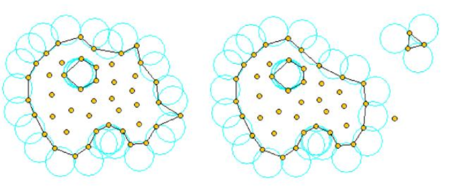Figure 2: Identification of individual particles (or a group of particles) starting from a given collection of nodes using the Alpha Shape method.

9 COMPUTATION OF THE CHARACTERISTIC LENGTHS

The evaluation of the stabilization parameters is one of the crucial issues in stabilized methods. Excellent results have been obtained in all problems solved using linear elements with the characteristic length vector defined by

 ${\boldsymbol {h}}=h_{s}{{\boldsymbol {u}} \over {u}}+h_{c}{{\boldsymbol {\nabla }}u \over \vert {\boldsymbol {\nabla }}u\vert }$
(59)

where ${\textstyle u=\vert {\boldsymbol {u}}\vert }$ and ${\textstyle h_{s}}$ and ${\textstyle h_{c}}$ are the “streamline” and “cross wind” contributions given by

 $h_{s}=\max({\boldsymbol {l}}_{j}^{T}{\boldsymbol {u}})/{u}$ (60) $h_{c}=\max({\boldsymbol {l}}_{j}^{T}{\boldsymbol {\nabla }}u)/\vert {\boldsymbol {\nabla }}u\vert \quad ,\quad j=1,n_{s}$ (61)

where ${\textstyle {\boldsymbol {l}}_{j}}$ are the vectors defining the element sides (${\textstyle n_{s}=6}$ for tetrahedra).

As for the free surface equation in the ALE formulation the following value of the characteristic length vector ${\textstyle {\boldsymbol {h}}_{\beta }}$ in Eq.(52) has been taken

 ${\boldsymbol {h}}_{\beta }={\bar {h}}_{s}{{\boldsymbol {u}} \over {u}}+{\bar {h}}_{c}{{\boldsymbol {\nabla }}\beta \over \vert {\boldsymbol {\nabla }}\beta \vert }$
(62)

The streamline parameter ${\textstyle {\bar {h}}_{s}}$ has been obtained by Eq.(60) using the value of the velocity vector ${\textstyle {\boldsymbol {u}}}$ over the 3 node triangles discretizing the free surface and ${\textstyle n_{s}=3}$.

The cross wind parameter ${\textstyle {\bar {h}}_{c}}$ has been computed by

 ${\bar {h}}_{c}=\max[{\boldsymbol {l}}_{j}^{T}{\boldsymbol {\nabla }}\beta ]{1 \over \vert {\boldsymbol {\nabla }}\beta \vert }\quad ,\quad j=1,2,3$
(63)

The cross-wind terms in eqs.(59) and (63) account for the effect of the gradient of the solution in the stabilization parameters. This is a standard assumption in most “shock-capturing” stabilization procedures .

10 EXAMPLES

The examples chosen show the applicability of the Eulerian, ALE and Lagrangian (PFEM) formulations presented to solve fluid flow problems. The first two examples are typical flow problems solved with the standard Eulerian formulation using the predictor-corrector scheme of Section 4.4 with ${\textstyle \theta =1}$. The first example is the flow of an inviscid fluid past a cylinder. Then the standard problem of flow past a NACA airfoil is solved with mesh adaptivity.

The next two examples fall within the category of ship hydrodynamics problems solved with the ALE formulation and the fractional step scheme of Section 4.2 with ${\textstyle \alpha =1}$. The examples are the analysis of a scale model of a commercial ship and of an American Cup racing sail boat. In both cases the free surface equation is solved together with the flow equations as described in Section 6. Numerical results are compared with experimental data.

The last series of examples show applications of the Lagrangian PFEM formulation to the simulation of the collapse of a water column, a sloshing problem, a ship hit by a wave, a solid cube falling into a water recipient and a mixing problem.

10.1 Inviscid flow past a cylinder

The problem was solved with the mesh of 10096 linear triangles shown in Figure 3. The initial conditions were an horizontal velocity of value one and zero pressure. A value of the Reynolds number of infinity was taken. The solution was progressed in time until steady state was found. The “correct” solution in this case is a symmetric velocity and pressure field.

The steady state results in Figure 4 show the relevance of accounting for the convective projection terms in the momentum equations. Results for the velocity and pressure contours shown in Figure 5 were obtained with the formulation described in the paper, whereas the results shown in Figure 6 were obtained by neglecting the convective projection terms (i.e. neglecting the terms involving the ${\textstyle {\boldsymbol {c}}}$ variables in Eq.(44a) and skipping the solution of Eq.(44c)). It can be clearly seen that neglecting the convective projection terms leads to a deterioration of the results in the region behind the cylinder where some oscillations for the pressure and velocity fields are found. These oscillations disappear and the correct symmetric solution is found if the convective projection terms are taken into account as described in the paper.

Evidence of the importance of taking into account the pressure gradient projection terms for the accuracy of the incompressible solution has also been found as reported in .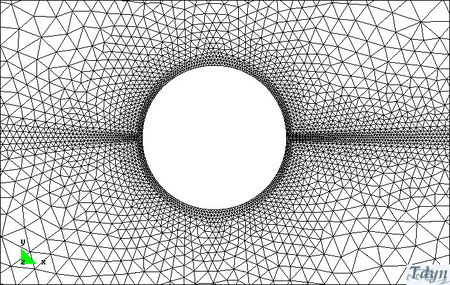Figure 3: Inviscid flow past a cylinder. Mesh of 10.096 three node triangles.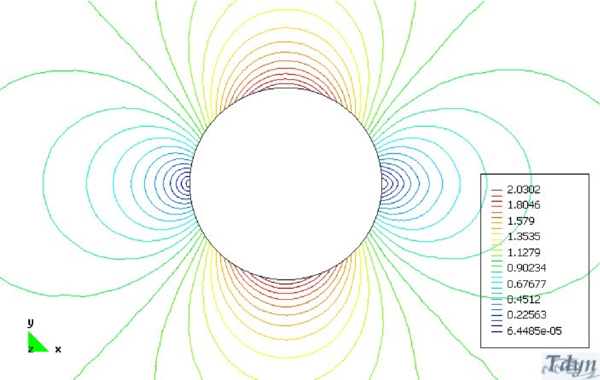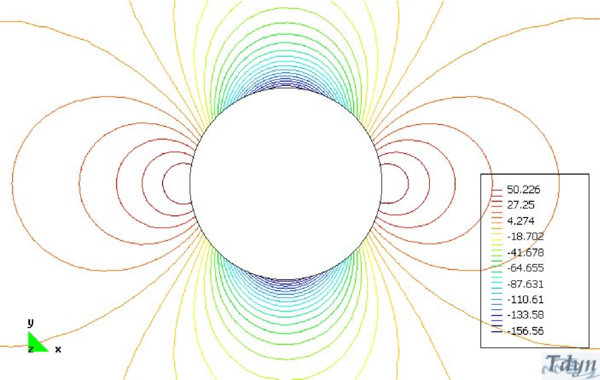Figure 4: Contours of velocity (upper picture and pressure) obtained accounting for the convective projection terms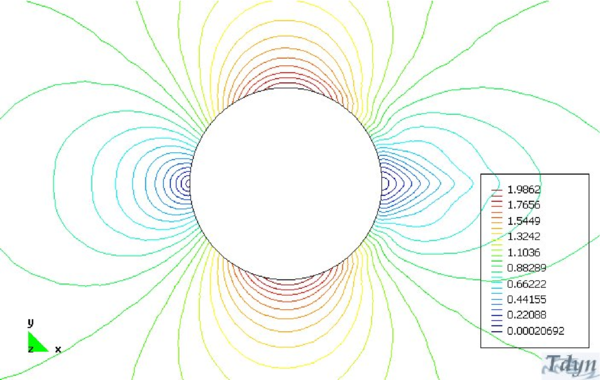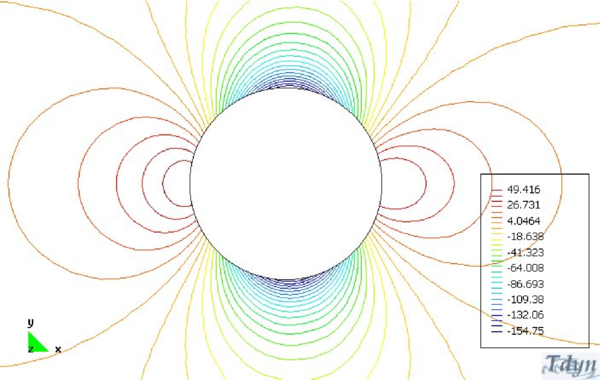Figure 5: Contours of velocity (upper picture and pressure) obtained not taking into account the convective projection terms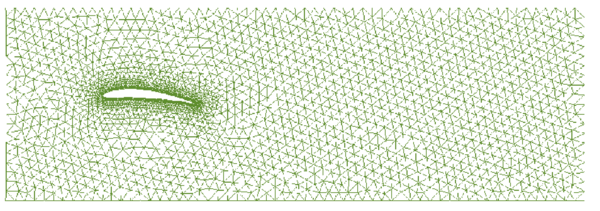Figure 6: Viscous flow past a NACA airfoil. Characteristic length =1m, $Re=100$. Original mesh

10.2 Flow past a NACA airfoil. Adaptative solution

The problem is the analysis of a viscous flow past a NACA 12 airfoil. This example sows the performance of the viscous FIC formulation with a mesh refinement scheme. The initial mesh of 2574 nodes and 4784 linear triangular elements is shown in Figure 6. The problem is solved for a value of the Reynolds number of 100.

The mesh refinement algorithm is based on the equidistribution of the global error over the finite element mesh. The error estimation method is based on the evaluation of the energy rate (the power) of the FE residuals of the momentum and the incompressibility equations. The residuals are computed using recovered values of the derivatives and pressure variables obtained via a nodal derivative recovery technique. A nodal-based approach is used for computing the residual power integrals . The refinement was performed at a time ${\textstyle t=2}$s during the transient solution process. The resultant mesh after five refinement steps is shown in Figures 7 and Figure 8. The corresponding velocity field contours are shown in Figures 9 and 10.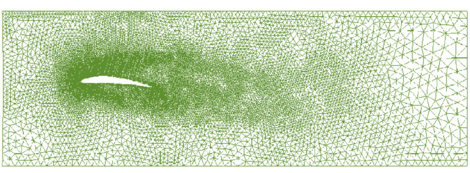Figure 7: Mesh obtained after five refinement steps using the equidistribution of the global error.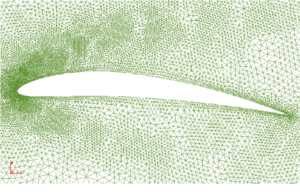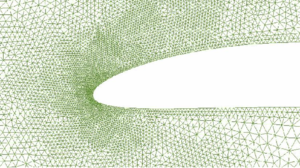Figure 8: Details of the refined mesh of Figure 7 in the vecinity of the airfoil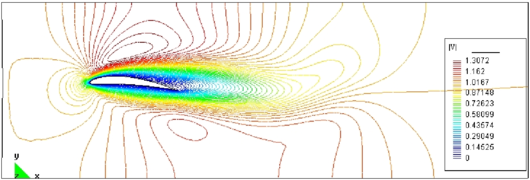Figure 9: Velocity field after five refinement stepsFigure 10: Detail of the velocity field of Figure 9

Table 1 shows the value of the global error computed as the percentage of the energy rate of the FE residuals (${\textstyle \Vert P\Vert _{\Omega }}$) versus the total energy rate ${\textstyle U}$, the errors in the drag and lift values and the number of elements and nodes during the mesh refinement process.

Further examples of mesh adaptivity in incompressible flows using the FIC formulation can be found in .

 Mesh Global error ${\textstyle \left({\Vert P\Vert _{\Omega } \over U}\right)}$(%) Nodes Elements Drag error % Lift error% 1 5.55 2574 4784 29.1810942 2.99902143 2 2.93 5340 10680 28.7443059 2.5500113 3 2.87 7942 15884 22.1078024 2.00851784 4 2.77 11948 23896 15.2893198 1.54898957 5 1.81 17142 34284 10.6478917 1.17033653 6 1.81 17255 34510 8.54878424 1.02567233 7 1.71 17372 34744 6.93290243 0.86514698

10.3 KVLCC2 hull model

The example is the analysis of the so called KVLCC2 benchmark model proposed by the Korean Research Institute of Ship and Ocean Engineering (KRISO) . Here a partially wetted tramsom stern is expected due to the low Froude number of the test. Figure 11 shows the NURBS geometry of the ship provided by KRISO. The obtained results are compared with the experimental data available .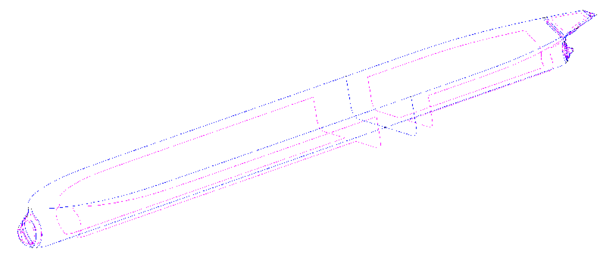Figure 11: KVLCC2 model. Geometrical definition based on NURBS surfaces.

The smallest element size used was 0.001 m and the largest 0.50 m. The surface mesh chosen is shown in Figure 12. A total of 550000 tetrahedra were used to model the virtual towing basin. The two following test cases were analyzed.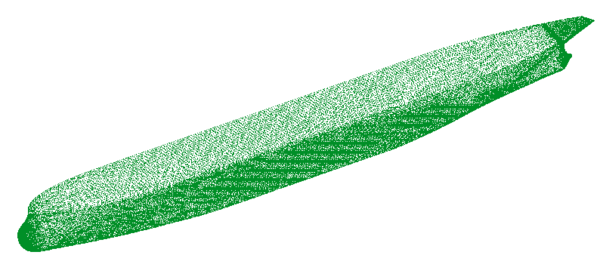Figure 12: KVLCC2 model. Surface mesh used in the analysis.

Test 1.- Wave pattern calculation. The main characteristics of the analysis are listed below:

• Length: 5.52 m, Beam (at water plane): 0.82 m, Draught: 0.18 m, Wetted Surface: ${\textstyle 8.08m^{2}}$.
• Velocity: 1.05 m/sec, Froude Number: 0.142.
• Viscosity: ${\textstyle 0.00126Kg/m\cdot sec}$, Density: ${\textstyle 1000Kg/m^{3}}$, Reynolds number: ${\textstyle 4.63\times 10^{6}}$.

The turbulence model chosen in this case was the ${\textstyle K}$ model. Figures 13 and 14 show the wave profiles on the hull and in a cut at y/L = 0.082 compared to the experimental data. The numerical results are quantitatively good close to the hull. A loss of accuracy is observed in the profiles away from the hull. This is probably due to the fact that the element sizes are not small enough in this area.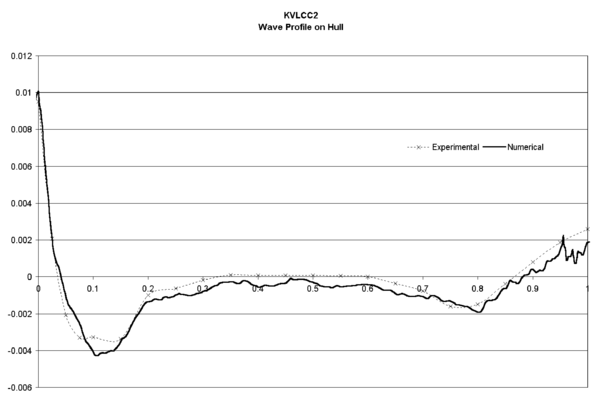Figure 13: KVLCC2 model. Wave profile on the hull compared to experimental data. Thick line shows numerical results

Test 2.- Wake analysis at different planes. Several turbulence models were used in order to verify the quality of the results. Here, only the results from the ${\textstyle K-\epsilon }$ model are shown. We note that the velocity maps obtained even for the simplest ${\textstyle Smagorinsky}$ model were qualitatively good, showing the accuracy of the fluid solver scheme used. The main characteristics of this analysis are:

• Length: 2.76 m, Beam (at water plane): 0.41 m, Draught: 0.09 m, Wetted Surface: ${\textstyle 2.02m^{2}}$.
• Velocity: 25 m/seg.
• Viscosity: ${\textstyle 3.05\cdot 10^{-5}Kg/m\cdot seg}$, Density: ${\textstyle 1.01Kg/m^{3}}$, Reynolds number: ${\textstyle 4.63\cdot 10^{6}}$.

Figures 15–16 present results corresponding to the test 2. Figure 15 shows the contours of the axial (X) component of the velocity on a plane at 2.71 m from the orthogonal aft. Figure 16 shows the maps of the kinetic energy on this plane. Experimental results are shown for comparison in all cases. Further results for this problem and other similar ones can be found in .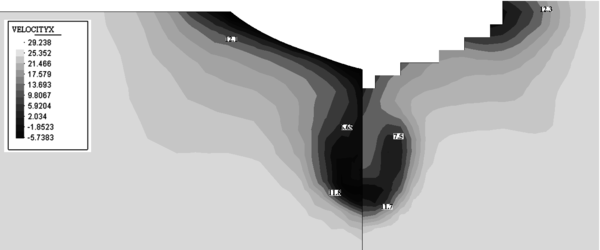Figure 15: KVLCC2 model. Map of the X component of the velocity on a plane at 2.71 m from the orthogonal aft. Experimental results shown in the right figure.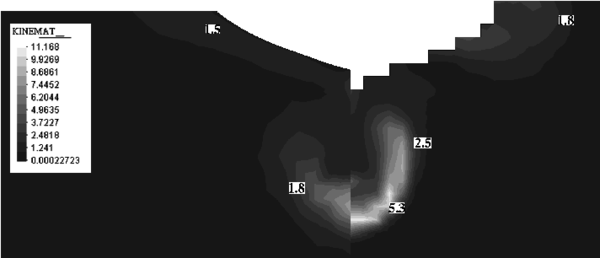Figure 16: KVLCC2 model. Map of the eddy kinetic energy ($K$) on a plane at 2.71 m from the orthogonal aft. Experimental data shown in the right figure.

10.4 American Cup BRAVO ESPAA Model

The next example is the analysis of the Spanish American Cup racing sail boat Bravo España. The finite element mesh used is shown in Figure 17. The numerical scheme is the same as that used for the previous example. The results presented in Figures 17–20 correspond to the analysis of a non symmetrical case including appendages. Good comparison between the experimental data and the numerical results was again obtained.

Other results of the hydrodynamic analysis of American Cup racing boats carried out with the FEM formulation presented in the paper can be seen in .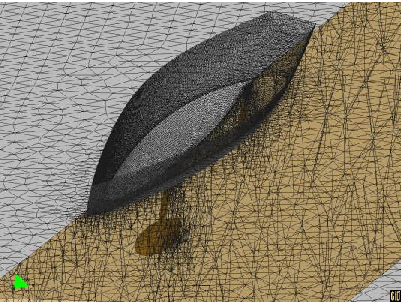Figure 17: $Bravo~Espa{\tilde {n}}a$ sail racing boat. Mesh used in the analysis.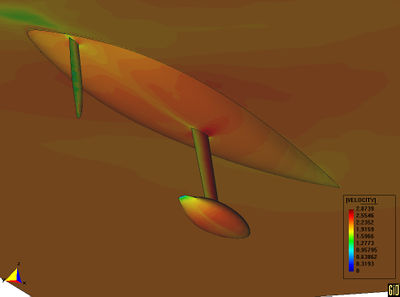Figure 18: $Bravo~Espa{\tilde {n}}a$. Velocity contours.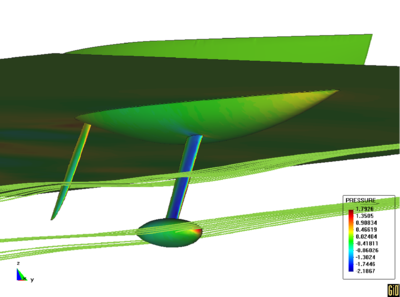Figure 19: $Bravo~Espa{\tilde {n}}a$. Streamlines.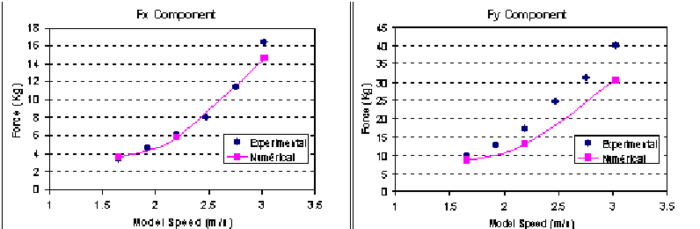Figure 20: $Bravo~Espa{\tilde {n}}a$. Resistance test. Comparison of numerical results with experimental data.

10.5 Collapse of a water column

The first problem solved to show the potential of the Lagrangian PFEM is the study of the collapse of a water column. This problem was solved by Koshizuka and Oka  both experimentally and numerically. It has became a classical example to validate the Lagrangian formulation for fluid flows. The water is initially kept within a rectangular container including a removable vertical board. A double layer of nodes in the solid walls is used in order to prevent water nodes from exiting the analysis domain. The boundary conditions impose zero velocity at the wall nodes and zero (atmospheric) pressure at the free surface. The method allows one to follow the large motion of the water particles including separation of some water drops. The collapse starts at time t = 0, when the board is removed. Viscosity and surface tension are neglected in the analysis. Figure 21 shows the point positions at different time steps. The dark points represent the free surface detected with the algorithm described in Section 8. The internal points are shown in a gray colour and the fixed points in black.

The water is running on the bottom wall until, at 0.3 sec it impinges on the right vertical wall. Breaking waves appear at 0.6 sec. At about 1 sec. the wave again reaches the left wall. Agreement with the experimental results  both in the shape of the free surface as well as in its time evolution are excellent.

Figure 22 shows the finite element mesh generated at a time step. We recall that this mesh is used to solve the equations of motion of the fluid particles as described in the previous sections.

The 3D solution of the same case is shown in Figure 23. More information on the PFEM solution of this problem can be found in .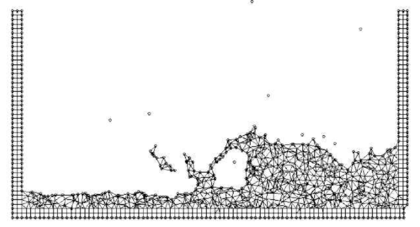Figure 22: Finite element mesh discretizing the fluid domain and the container walls at a certain time step.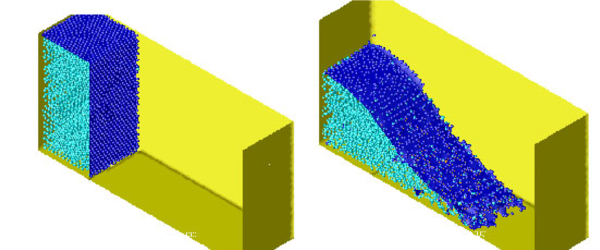(a) t = 0 sec. (b) t = 0.2 sec.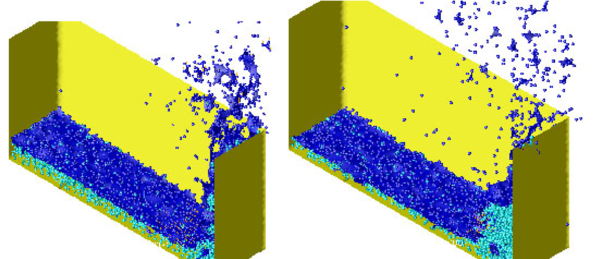(c) t = 0.4 sec. (d) t = 0.6 sec.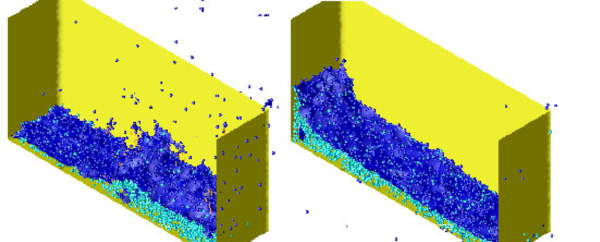(e) t = 0.8 sec. (f) t = 1.1 sec. Figure 23: Water column collapse in a 3D domain.

10.6 Sloshing problems

The simple problem of the free oscillation of an incompressible liquid in a container is considered next. A numerical and analytical solution for this problem can be found in . Figure 24 shows a schematic view of the problem and the point distribution in the initial position. The dark points represent the fixed points on the walls where the velocity is fixed to zero.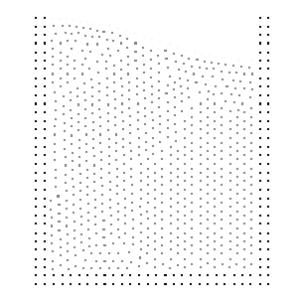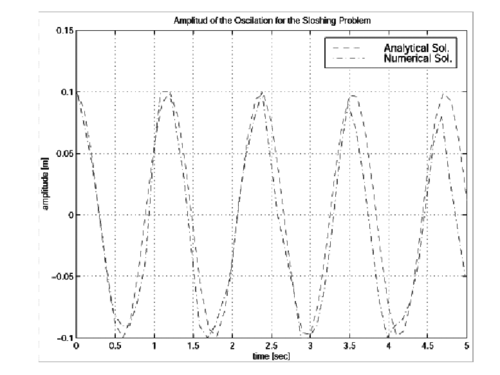Figure 24: Sloshing. Initial point distribution and comparison of the numerical and analytical solutions.

Figure 24 also shows the time evolution of the amplitude compared with the analytical results for the near inviscid case. Little numerical viscosity is observed on the phase wave and amplitude in spite of the relative coarse distribution of nodes.

The analytical solution is only acceptable for small wave amplitudes. For larger amplitudes, additional waves are overlapping and, finally, the wave breaks and also some particles separate from the fluid domain due to their large velocity. Figure 25 shows the numerical results obtained with the PFEM for this case. Breaking waves as well as separation effects can be seen on the free surface. This particular and very complicated effect is well represented by the PFEM.

In order to further test the potential of the PFEM the same sloshing problem was solved in 3D. Figure 26 shows the different point positions at two time steps. Each point position was represented by a sphere and only a half of the fixed recipient is represented on the figure. This representation is only used in order to improve the visualization of the numerical results.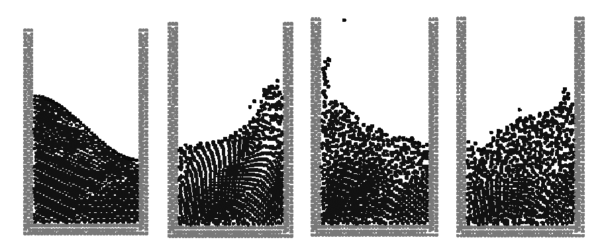Figure 25: PFEM results for a large amplitude sloshing problem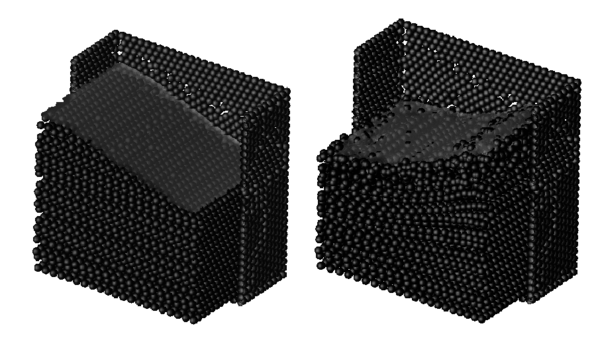Figure 26: 3D sloshing problem

10.7 Container ship hit by an incoming wave

Figure 27 shows the analysis of the motion of the transverse sections of a container ship hit by an incoming wave. The dynamic motion of the ship is induced by the resultant of the pressure and the viscous forces acting on the ship boundaries. The section of the ship analyzed corresponds to that of a real container ship. The ship is assumed to be rigid and is free to move laterally due to the sea wave forces. The objective of the study was to asses the influence of the stabilizers in the ship roll. The figures show clearly how the PFEM predicts the ship and wave motions in a realistic manner. Further examples of this type solved with the PFEM can be found in .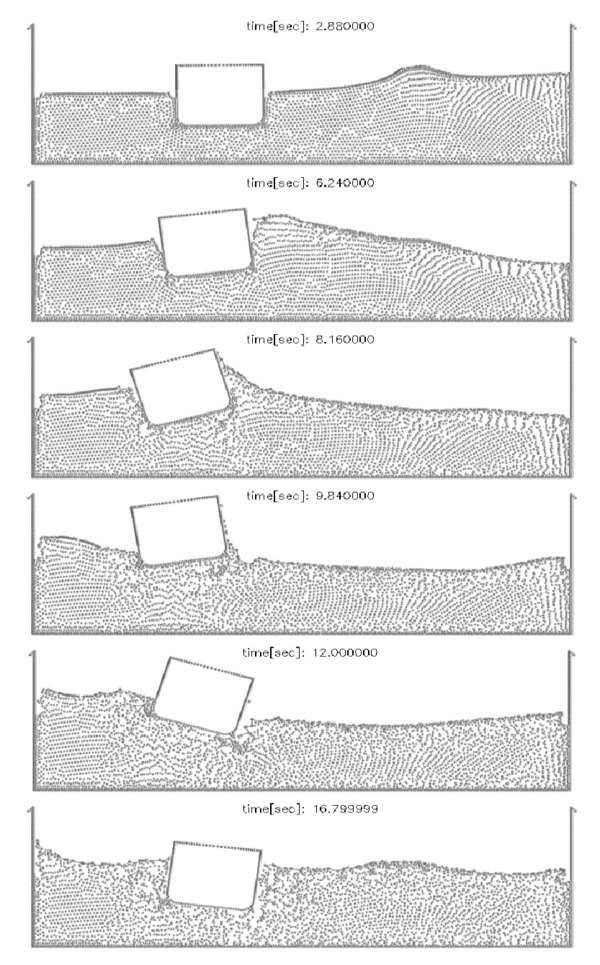Figure 27: Ship with stabilizers hit by a lateral wave

10.8 Rigid square falling in a recipient with water

In the next example a solid square is initially free and falls down within a water recipient. The square is modelled a rigid solid subjected to pressure and viscous forces acting in its boundaries. The resultant of the fluid forces and the weight of the square are applied to the center of the square. These forces govern the displacement of the square which is computed by solving the dynamic equations of motion as described in the fractional step algorithm of Section 6, similarly as for the rigid ship of the previous example. Here again the moving square contours define a boundary condition for the fluid particles at each time step.

Initially the solid falls down freely due to the gravity forces (Figure 28). Once in contact with the water surface, the Alpha-Shape method recognizes the different boundary contours which are shown with a thick line in the figure. The pressure and viscous forces are evaluated in all the domain and in particular on the square contours. The fluid forces introduce a negative acceleration in the vertical motion until, once the square is completely inside the water, the vertical velocity becomes zero. Then, buoyancy forces bring the square up to the free-surface. It is interesting to observe that there is a rotation of the square. The reason is that the center of the floating forces is higher in the rotated position than in the initial ones.

Figure 29 shows a repetition of the same problem showing now all the finite elements in the mesh discretizing the fluid. We recall that in all the problems here described the mesh in the fluid domain is regenerated at each time step combining linear triangles and quadrilateral elements as described in Section 8.3. Note that some fluid particles separate from the fluid domain. These particles are treated as free boundary points with zero pressure and hence fall down due to gravity.

It is interesting to see that the final position of the square is different from that of Figure 28. This is due to the unstable character of the square motion. A small difference in the numerical computations (for instance in the mesh generation process) shifts the movement of the square towards the right or the left. Note that a final rotated equilibrium position is found in both cases. For further details see .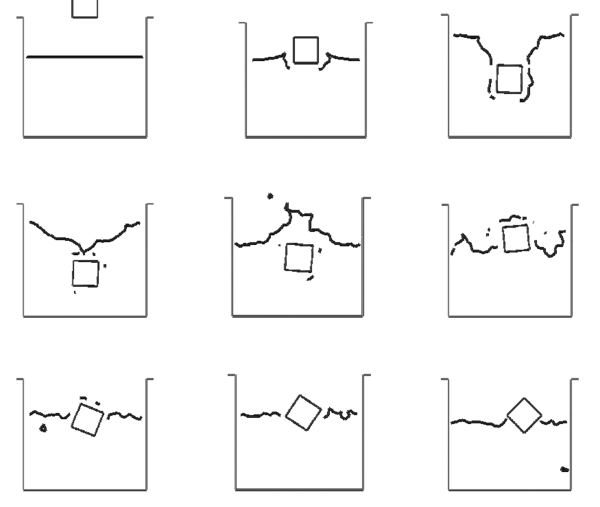Figure 28: Square falling into a recipient with water. The square is modelled as a rigid solid. Motion of the square and free surface positions at different time steps.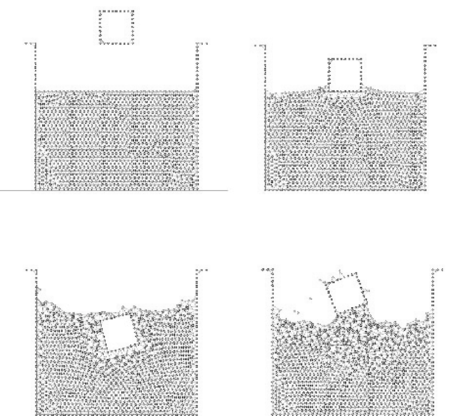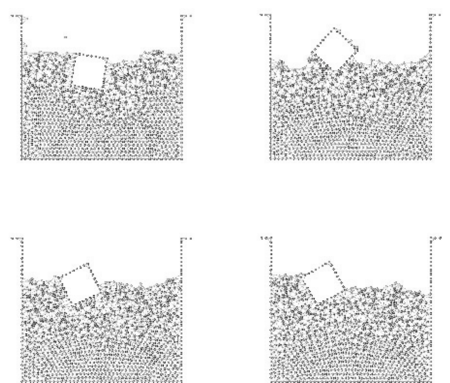Figure 29: Square falling in a water recipient. The square is modeled as a rigid solid. The finite element meshes generated at the selected instants are shown.

10.9 Mixing of particles within a fluid

Figure 30 shows an example of application of the PFEM to the mixing of a collection of particles within a container containing a fluid of a higher density. Initially the particles are thrown into the container and mix with the fluid as shown. As time evolves the particles move up naturally towards the surface of the fluid due to their smaller density. This example clearly shows the possibilities of the PFEM for analysis of fluid mixing situations.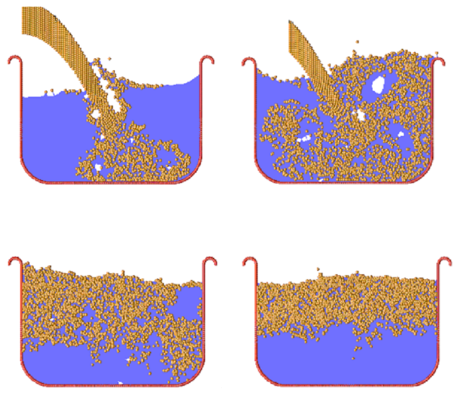Figure 30: Mixing of particles in a fluid. Evolution of the particles during the mixing process

11 CONCLUSIONS

The finite calculus form of the fluid mechanics equations is a good starting point for deriving stabilized finite algorithms for solving a variety of fluid flow problems using Euler, ALE and fully Lagrangian descriptions. Fractional step and predictor-corrector algorithms with intrinsic stabilization properties can be readily derived from the FIC governing equations. Free surface wave effects and fluid-structure interaction situations can be accounted for in a straightforward manner within the general flow solution algorithm. The ALE formulation is particularly adequate for analysis of problems involving free surface waves of moderate amplitude, typical of ship hydrodynamics situations. The Lagrangian PFEM formulation is very effective for fluid flow problems involving large motions of the free surface, splashing of waves, complex fluid-structure interactions and mixing problems.

ACKNOWLEDGEMENTS

The authors are grateful to Copa America Desafio Español SA for providing the geometry and experimental data of the racing boat analyzed in example 10.4.

Examples 10.1–10.4 were analyzed with the finite element code Tdyn based on the FEM formulation here presented .

Thanks are also given to Profs. R.L. Taylor and R. Löhner, Dr. R. Flores and Mr. R. Aubry for many useful discussions.

BIBLIOGRAPHY

 O.C. Zienkiewicz and R.C. Taylor,The finite element method, 5th Edition, 3 Volumes, Butterworth–Heinemann, 2000.

 C. Hirsch, Numerical computation of internal and external flow, J. Wiley, Vol. 1 1988, Vol. 2, 1990.

 A.J. Chorin, “A numerical solution for solving incompressible viscous flow problems” J. Comp. Phys., 2, 12–26, 1967.

 W.R. Briley, S.S. Neerarambam and D.L. Whitfield, “Multigrid algorithm for three-dimensional incompressible high-Reynolds number turbulent flows”, AIAA Journal, 33 (1), 2073–2079, 1995.

 J. Peraire, K. Morgan and J. Peiro, “The simulation of 3D incompressible flows using unstructured grids”, In Frontiers of Computational Fluid Dynamics, Caughey DA and Hafez MM. (eds), Chapter 16, J. Wiley, 1994.

 C. Sheng, L.K. Taylor and D.L. Whitfield, “Implicit lower-upper/approximate-factorization schemes for incompressible flows” Journal of Computational Physics, 128 (1), 32–42, 1996.

 M. Storti, N. Nigro and S.R. Idelsohn, “Steady state incompressible flows using explicit schemes with an optimal local preconditioning”, Computer Methods in Applied Mechanics and Engineering, 124, 231–252, 1995.

 J.C. Heinrich, P.S. Hayakorn and O.C. Zienkiewicz, “An upwind finite element scheme for two dimensional convective transport equations”, Int. J. Num. Meth. Engng., 11, 131–143, 1977.

 S.R. Idelsohn, M. Storti and N. Nigro, “Stability analysis of mixed finite element formulation with special mention to stabilized equal-order interpolations” Int. J. for Num. Meth. in Fluids, 20, 1003-1022, 1995.

 S.R. Idelsohn, N. Nigro, M. Storti and G. Buscaglia, “A Petrov-Galerkin formulation for advection-reaction-diffusion”, Comput. Meth. Appl. Mech. Engrg., 136, 27–46, 1996.

 A. Brooks and T.J.R. Hughes, “Streamline upwind/Petrov-Galerkin formulation for convection dominated flows with particular emphasis on the incompressible Navier-Stokes equations”, Comput. Methods Appl. Mech. Engrg, 32, 199–259, 1982.

 T.J.R. Hughes and M. Mallet, “A new finite element formulations for computational fluid dynamics: III. The generalized streamline operator for multidimensional advective-diffusive systems”, Comput Methods Appl. Mech. Engrg., 58, pp. 305–328, 1986.

 P. Hansbo and a. Szepessy, “A velocity-pressure streamline diffusion finite element method for the incompressible Navier-Stokes equations”, Comput. Methods Appl. Mech. Engrg., 84, 175–192, 1990.

 T.J.R. Hughes, L.P. Franca and M. Balestra, “A new finite element formulation for computational fluid dynamics. V Circumventing the Babuska-Brezzi condition: A stable Petrov-Galerkin formulation of the Stokes problem accomodating equal order interpolations”, Comput. Methods Appl. Mech. Engrg., 59, 85–99, 1986.

 L.P. Franca and S.L. Frey, “Stabilized finite element methods: II. The incompressible Navier-Stokes equations”, Comput. Method Appl. Mech. Engrg., Vol. 99, pp. 209–233, 1992.

 T.J.R. Hughes, G. Hauke and K. Jansen, “Stabilized finite element methods in fluids: Inspirations, origins, status and recent developments”, in: Recent Developments in Finite Element Analysis. A Book Dedicated to Robert L. Taylor, T.J.R. Hughes, E. Oñate and O.C. Zienkiewicz (Eds.), (International Center for Numerical Methods in Engineering, Barcelona, Spain, pp. 272–292, 1994.

 M.A. Cruchaga and E. Oñate, “A finite element formulation for incompressible flow problems using a generalized streamline operator”, Comput. Methods in Appl. Mech. Engrg., 143, 49–67, 1997.

 M.A. Cruchaga and E. Oñate, “A generalized streamline finite element approach for the analysis of incompressible flow problems including moving surfaces”, Comput. Methods in Appl. Mech. Engrg., 173, 241–255, 1999.

 T.J.R. Hughes, L.P. Franca and G.M. Hulbert, “A new finite element formulation for computational fluid dynamics: VIII. The Galerkin/least-squares method for advective-diffusive equations”, Comput. Methods Appl. Mech. Engrg., 73, pp. 173–189, 1989.

 T.E. Tezduyar, S. Mittal, S.E. Ray and R. Shih, “Incompressible flow computations with stabilized bilinear and linear equal order interpolation velocity–pressure elements”, Comput. Methods Appl. Mech. Engrg., 95, 221–242, 1992.

 J. Donea, “A Taylor-Galerkin method for convective transport problems”, Int. J. Num. Meth. Engng., 20, 101–119, 1984.

 J. Douglas, T.F. Russell, “Numerical methods for convection dominated diffusion problems based on combining the method of characteristics with finite element or finite difference procedures”, SIAM J. Numer. Anal., 19, 871, 1982.

 O. Pironneau, “On the transport-diffusion algorithm and its applications to the Navier-Stokes equations”, Numer. Math., 38, 309, 1982.

 R. Löhner, K. Morgan, O.C. Zienkiewicz, “The solution of non-linear hyperbolic equation systems by the finite element method”, Int. J. Num. Meth. in Fluids, 4, 1043, 1984.

 R. Codina, M. Vazquez and O.C. Zienkiewicz, “A general algorithm for compressible and incompressible flow - Part III. The semi-implicit form” Int. J. Num. Meth. in Fluids, 27, 13–32, 1998.

 R. Codina and O.C. Zienkiewicz, “CBS versus GLS stabilization of the incompressible Navier-Stokes equations and the role of the time step as stabilization parameter”, Communications in Numerical Methods in Engineering, 18 (2), 99–112, 2002.

 R. Codina and J. Blasco, “Stabilized finite element method for the transient Navier-Stokes equations based on a pressure gradient operator”, Comput. Methods in Appl. Mech. Engrg., 182, 277–301, 2000.

 T.J.R. Hughes, “Multiscale phenomena: Green functions, subgrid scale models, bubbles and the origins of stabilized methods”, Comput. Methods Appl. Mech. Engrg, Vol. 127, pp. 387–401, 1995.

 F. Brezzi, L.P. Franca, T.J.R. Hughes and A. Russo, $b=\int g$, Comput. Methods Appl. Mech. Engrg., 145, 329–339, 1997.

 R. Codina, “Stabilized finite element approximation of transient incompressible flows using orthogonal subscales”, Comput. Methods Appl. Mech. Engrg., 191, 4295–4321, 2002.

' J. Donea and A. Huerta, Finite element method for flow problems', J. Wiley, 2003.

 E. Oñate, Derivation of stabilized equations for advective-diffusive transport and fluid flow problems, Comput. Meth. Appl. Mech. Engng., Vol. 151, pp. 233–267, (1998).

 E. Oñate, J. García and S. Idelsohn, Computation of the stabilization parameter for the finite element solution of advective-diffusive problems, Int. J. Num. Meth. Fluids, Vol. 25, pp. 1385–1407, (1997).

 E. Oñate, J. García and S. Idelsohn, An alpha-adaptive approach for stabilized finite element solution of advective-diffusive problems with sharp gradients, New Adv. in Adaptive Comp. Met. in Mech., P. Ladeveze and J.T. Oden (Eds.), Elsevier, (1998).

 E. Oñate and M. Manzan, “A general procedure for deriving stabilized space-time finite element methods for advective-diffusive problems”, Int. J. Num. Meth. Fluids, 31, 203–221, 1999.

 E. Oñate and M. Manzan, “Stabilization techniques for finite element analysis of convection diffusion problems”, in Computational Analysis of Heat Transfer, G. Comini and B. Sunden (Eds.), WIT Press, Southampton, 2000.

 E. Oñate, “A stabilized finite element method for incompressible viscous flows using a finite increment calculus formulation”, Comp. Meth. Appl. Mech. Engng., 182, 1–2, 355–370, 2000.

 E. Oñate and J. García, “A finite element method for fluid-structure interaction with surface waves using a finite calculus formulation”, Comput. MethodsAppl. Mech. Engrg., 191, 635–660, (2001).

 E. Oñate, “Possibilities of finite calculus in computational mechanics”, Int. J. Num. Meth. Engng., 60(1), 255–281, 2004.

 J. García and E. Oñate, “An unstructured finite element solver for ship hydrodynamic problems”, Journal of Applied Mechanics, 70, 18–26, January 2003.

 E. Oñate, J. García and S.R. Idelsohn, “Ship hydrodynamics”, in Encyclopedia of Computational Mechanics, E. Stein, R. de Borst and T.J.R. Hughes (Eds), J. Wiley, 2004.

 E. Oñate and S. Idelsohn, A mesh free finite point method for advective-diffusive transport and fluid flow problems, Computational Mechanics, 21, 283–292, 1998.

 E. Oñate, C. Sacco and S. Idelsohn, “A finite point method for incompressible flow problems”, Computing and Visualization in Science, 2, 67–75, 2000.

 S.R. Idelsohn, E. Oñate, F. Del Pin and N. Calvo, “Lagrangian formulation: the only way to solve some free-surface fluid mechanics problems”, Fifth World Congress on Computational Mechanics, Mang HA, Rammerstorfer FG and Eberhardsteiner J. (eds.), July 7–12, Viena, Austria, 2002.

 S.R. Idelsohn, E. Oñate and F. Del Pin, “A lagrangian meshless finite element method applied to fluid-structure interaction problems”, Computer and Structures, 81, 655–671, 2003.

 E. Oñate, S.R. Idelsohn, F. Del Pin and R. Aubry, “The particle finite element method. An overview”, Submitted to Int. J. Comput. Meth., 1 (2), 2004.

 R. Aubry, S.R. Idelsohn and E. Oñate, “Particle finite element method in fluid mechanics including thermal convection-diffusion”. Computer & Structures, submitted, 2004.

 S.R. Idelsohn, E. Oñate and F. Del Pin, “The Particle Finite Element Method: a powerful tool to solve incompressible flows with free-surfaces and breaking waves”, Int. J. Num. Meth. Engng., (Submitted), 2004.

 S.R. Idelsohn, E. Oñate, N. Calvo and F. del Pin, “The meshless finite element method”, Int. J. Num. Meth. Engng., Vol. 58, pp. 893- 912, 2003.

 N. Calvo, S.R. Idelsohn and E. Oñate, “The extended Delaunay tesselation”, Engineering Computations, 20 (5/6), 583–600, 2003.

 S.R. Idelsohn, N. Calvo and E. Oñate, “Polyhedrization of an arbitrary 3D point set”, Comput. Meth. Appl. Mech. Engng., 192, 2649–2667, 2003.

 R. Codina, “Pressure stability in fractional step finite element method for incompressible flows”, J. Comput. Physics, 170, 112–140, 2001.

 R. Codina and O. Soto, “Approximation of the incompressible Navier-Stokes equations using orthogonal-subscale stabilization and pressure segregation on anisotropic finite element meshes”, Comput. Meth. Appl. Mech. Eng., to appear.

 T.E. Tezduyar, M. Behr and J. Liou, “A new strategy for finite element computations involving moving boundaries and interfaces - the deforming-spatial-domain/space-time procedure: I. The concept and the preliminary tests”, Comput. Methods in Appl. Mech. and Engrg., 94, 339–351, 1992.

 G. Chiandusi, G. Bugeda and E. Oñate, “A simple method for update of finite element meshes”, Commun, Numer. Meth. Engng., 16, 1–9, 2000.

 D.C. Wilcox, Turbulence modeling for CFD, DCW Industries Inc., 1994.

 H. Edelsbrunner and E.P. Mucke, E.P., “Three dimensional alpha shapes”, ACM Trans. Graphics, 13, 43–72, 1999.

 T.J.R. Hughes and M. Mallet, A new finite element formulations for computational fluid dynamics: IV. A discontinuity capturing operator for multidimensional advective-diffusive system, Comput. Methods Appl. Mech. Engrg., 58, 329–336, 1986.

 R. Codina, “A discontinuity-capturing crosswind dissipation for the finite element solution of the convection-diffusion equation”, Comput. Methods Appl. Mech. Engrg., 110, 325–342, 1993.

 E. Oñate, J. Rojek, R.L. Taylor and O.C. Zienkiewicz, “Finite calculus formulation for incompressible solids using linear triangles and tetrahedra”, Int. J. Num. Meth. Engng., 59, 1473–1500, 2004.

 E. Oñate, R.L. Taylor, O.C. Zienkiewicz and J. Rojek, “A residual correction method based on finite calculus”, Engineering Computations, 20, (5/6), 629–658, 2003.

 J.C. Cante, J. Oliver and C. González, “Powder forming simulation with the particle finite element method”, Computational Mechanics, Z.H. Yao, M.W. Yuan and W.X. Zhong (Eds.), CD-Rom Proceedings of the WCCM VI, Beijing, September 5–10, 2004.

 E. Oñate, J. Arteaga, J, García and R. Flores, “Error estimation and mesh adaptivity in incompressible viscous flows using a residual power approach”, Submitted to Comput. Meth. Appl. Mech. Engrg., 2004.

 Korea Research Institute of Ships and Ocean Engineering (KRISO).http://www.iihr.uiowa.edu/gothenburg2000/KVLCC/tanker.html.

 R. Löhner, C. Yang, E. Oñate and S. Idelsohn, An unstructured grid-based parallel free surface solver, Appl. Num. Math., 31, 271–293, (1999).

 J. García, R. Luco-Salman, M. Salas, M. López-Rodríguez and E. Oñate E, An advanced finite element method for fluid-dynamic analysis of America's Cup boat, High Performance Yacht Design Conference, Auckland, 4–6, December, 2002.

 S.R. Idelsohn, E. Oñate and C. Sacco, Finite element solution of the free surface ship-wave problem, Int. J. Num. Meth. Engng., 45, 503–508, 1999.

 S. Koshizuka and Y. Oka, “Moving particle semi-implicit method for fragmentation of incompressible fluid”, Nuclear Engineering Science, 123, 421-434, 1996.

 R. Radovitzki and M. Ortiz, “Lagrangian finite element analysis of a Newtonian flows”, Int. J. Num. Meth. Engng., 43, 607–619, 1998.

 Tdyn. A finite element code for fluid-dynamic analysis, COMPASS Ingeniería y Sistemas SA, www.compassis.com, 2004.

Document informationPublished on 11/04/19

DOI: 10.1016/j.cma.2004.10.016
Licence: CC BY-NC-SA license

Document Score0

Times cited: 41
Views 20
Recommendations 0